# NCERT Exemplar Class 9 Maths Solutions for Chapter 2 - Polynomials

## NCERT Exemplar Solutions Class 9 Maths Chapter 2 – Free PDF Download

NCERT Exemplar Class 9 Maths Chapter 2 Polynomials is provided here for students to prepare for exams. Students can use these materials as a reference tool for studying as it contains solved questions relevant to the exercise problems present in the NCERT Exemplar textbook. The exemplar problems are designed by experts in accordance with CBSE Syllabus for 9th standard, which covers the following topics of Chapter Polynomials given below:

• Finding the degree of polynomials
• Polynomials in one variable
• Coefficients and zeroes of polynomials
• Remainder theorem for polynomials
• Division of one polynomial from another
• The factorization of polynomials using Factor theorem
• Algebraic Identities to factorize polynomials

Before solving the questions let us learn what polynomial is. A polynomial is an expression of algebraic terms(more than two), especially the sum of several terms that contain different powers of the same variable. To facilitate easy learning and help students understand the concepts of polynomials, free NCERT Exemplars are provided here which can be further downloaded in the form of a PDF. Practice questions related to polynomials by solving the NCERT Exemplar for Class 9 Maths Chapter 2 Polynomials. To download pdf file of these materials click on the below link.

## Download PDF of NCERT Exemplar Solutions for Class 9 Maths Chapter 2 – Polynomials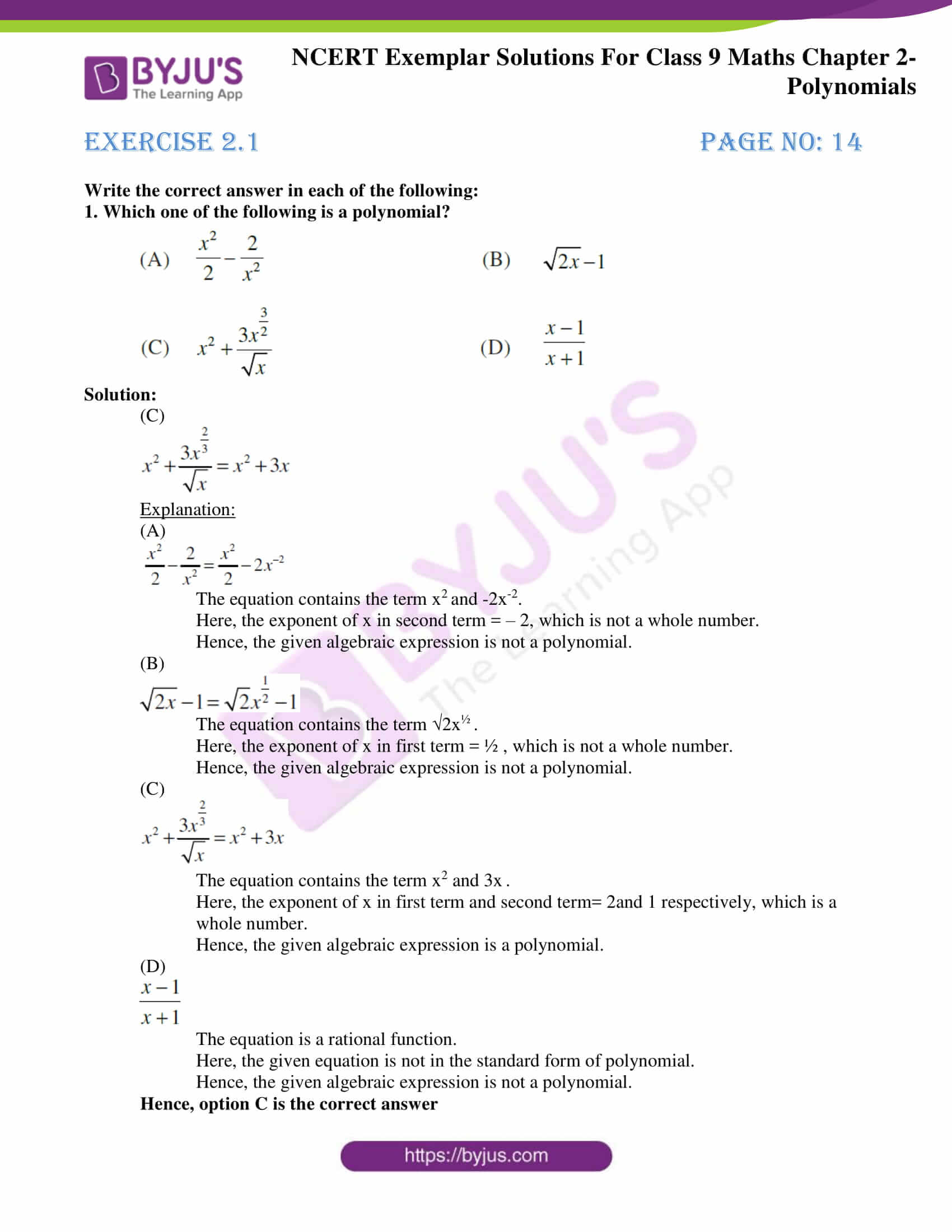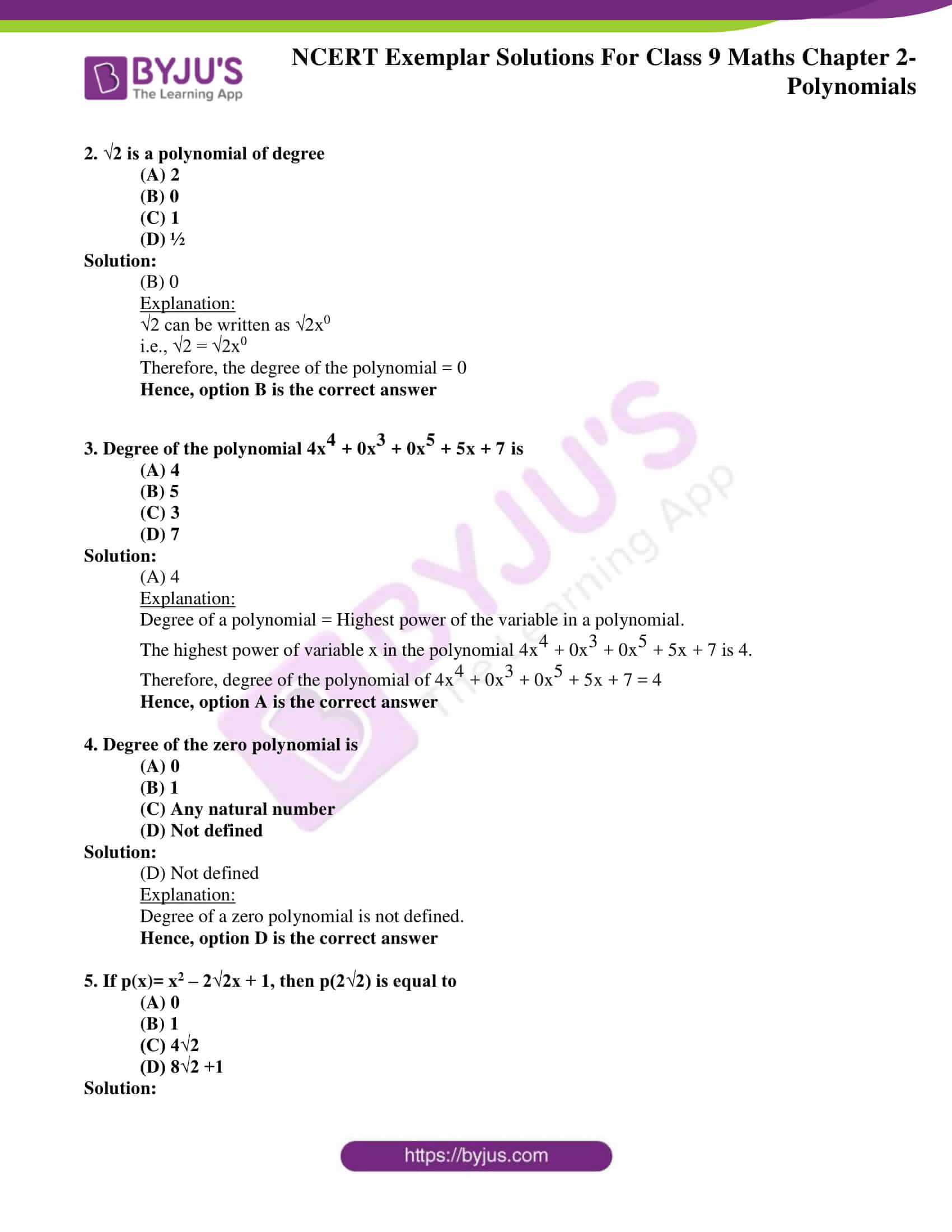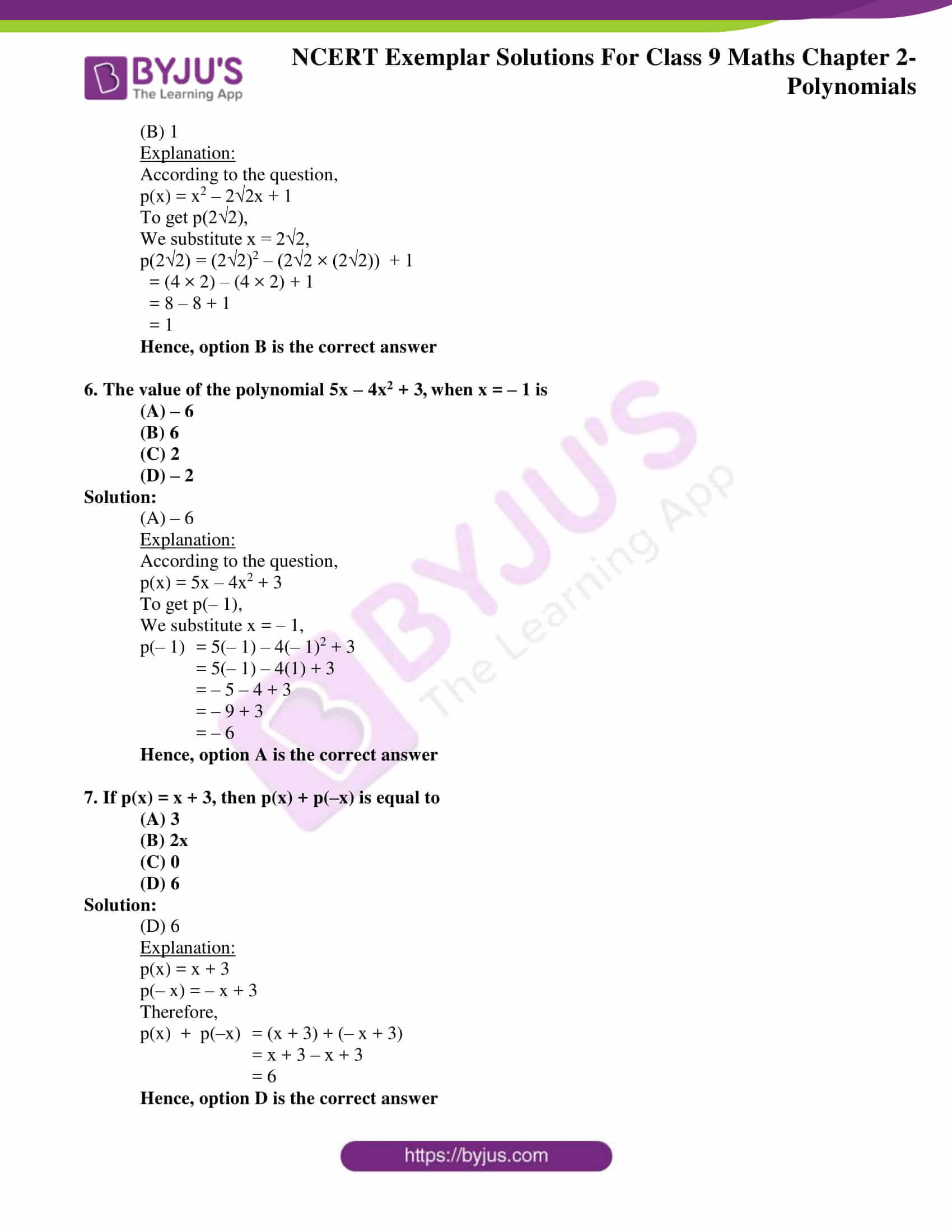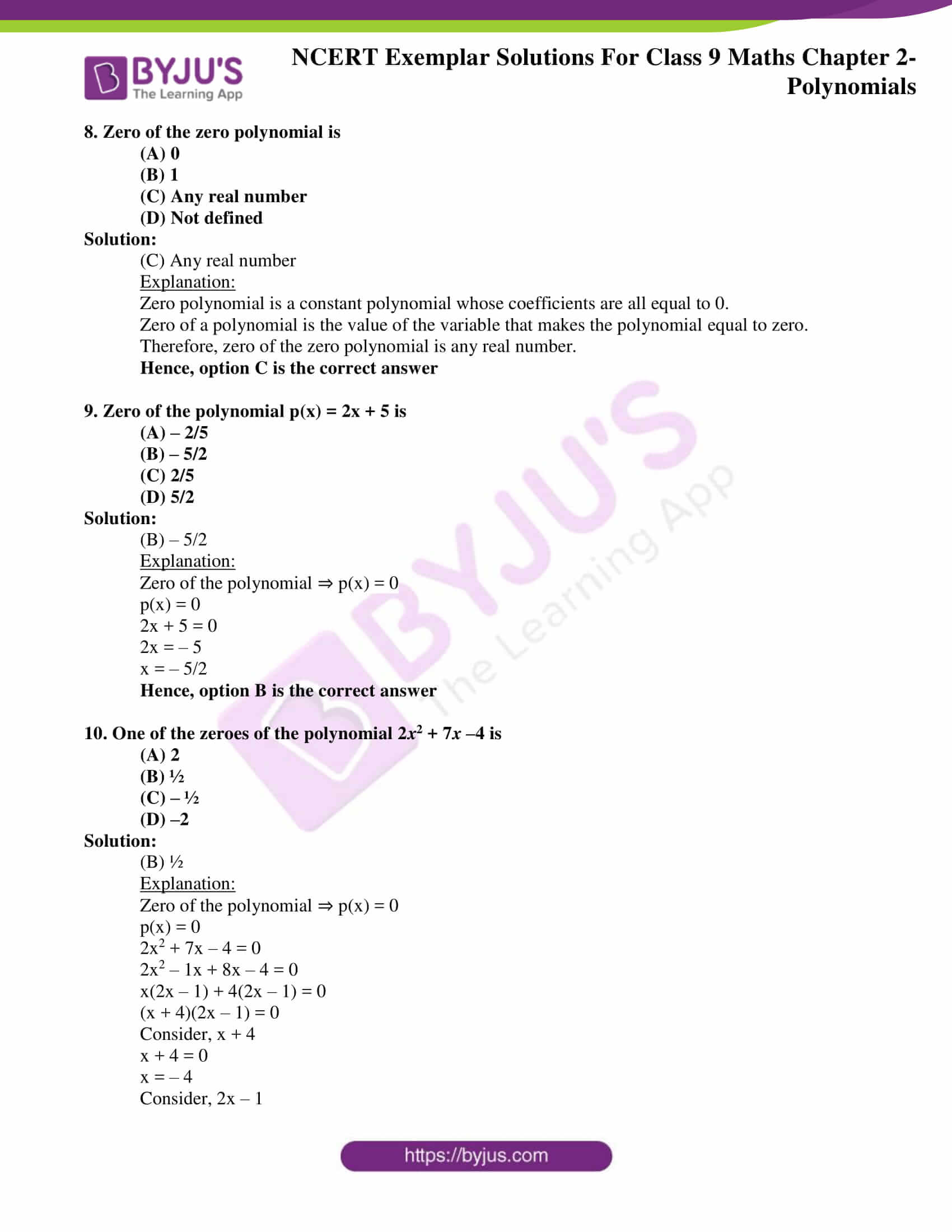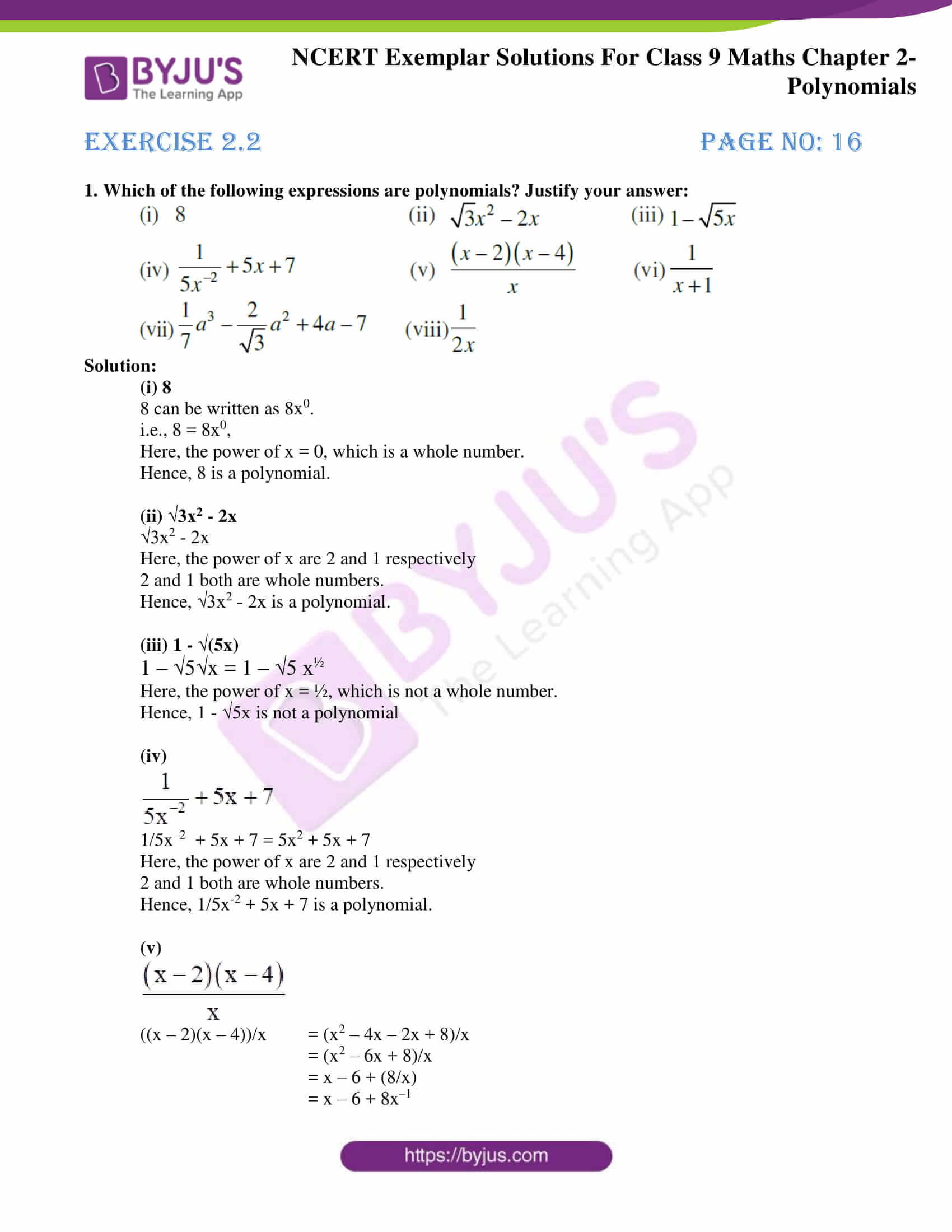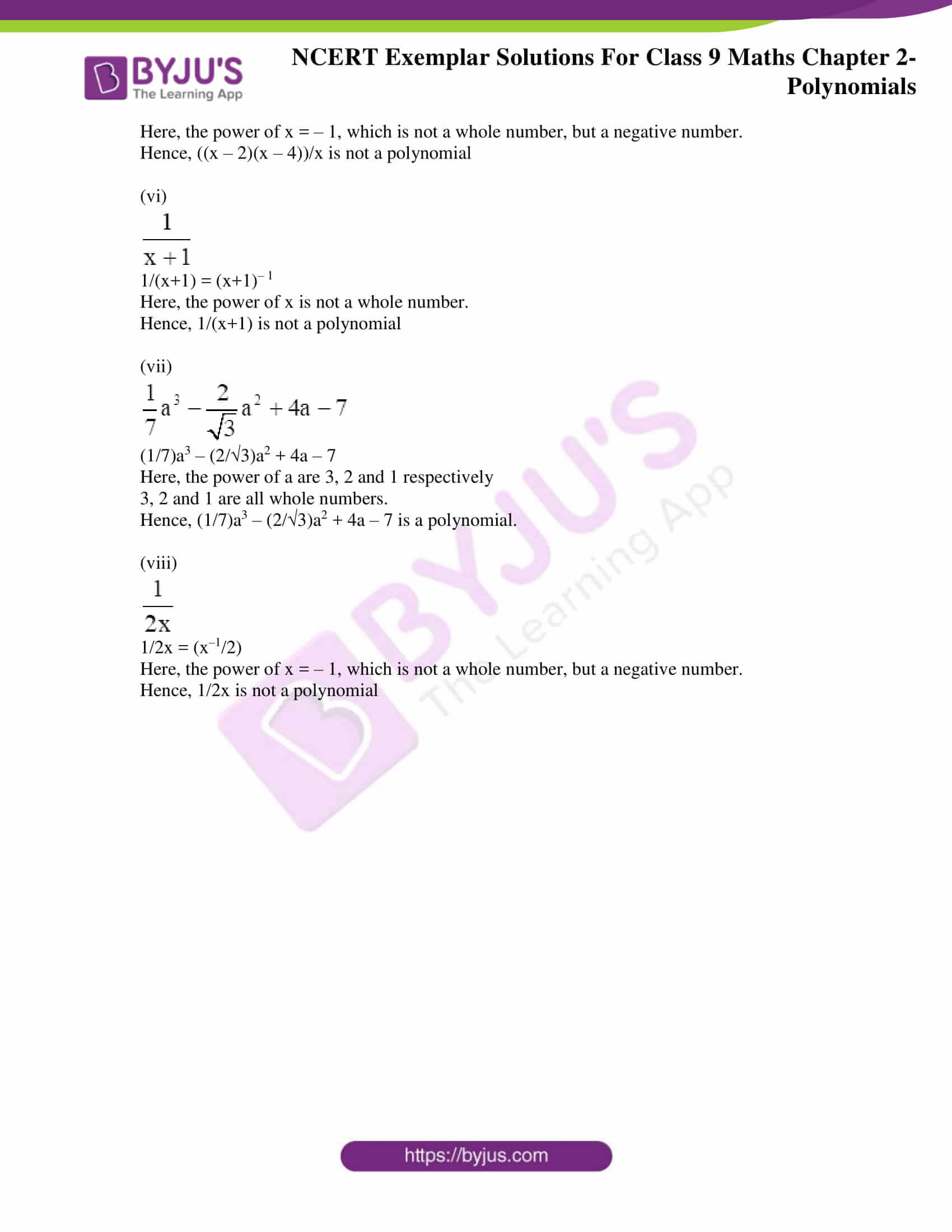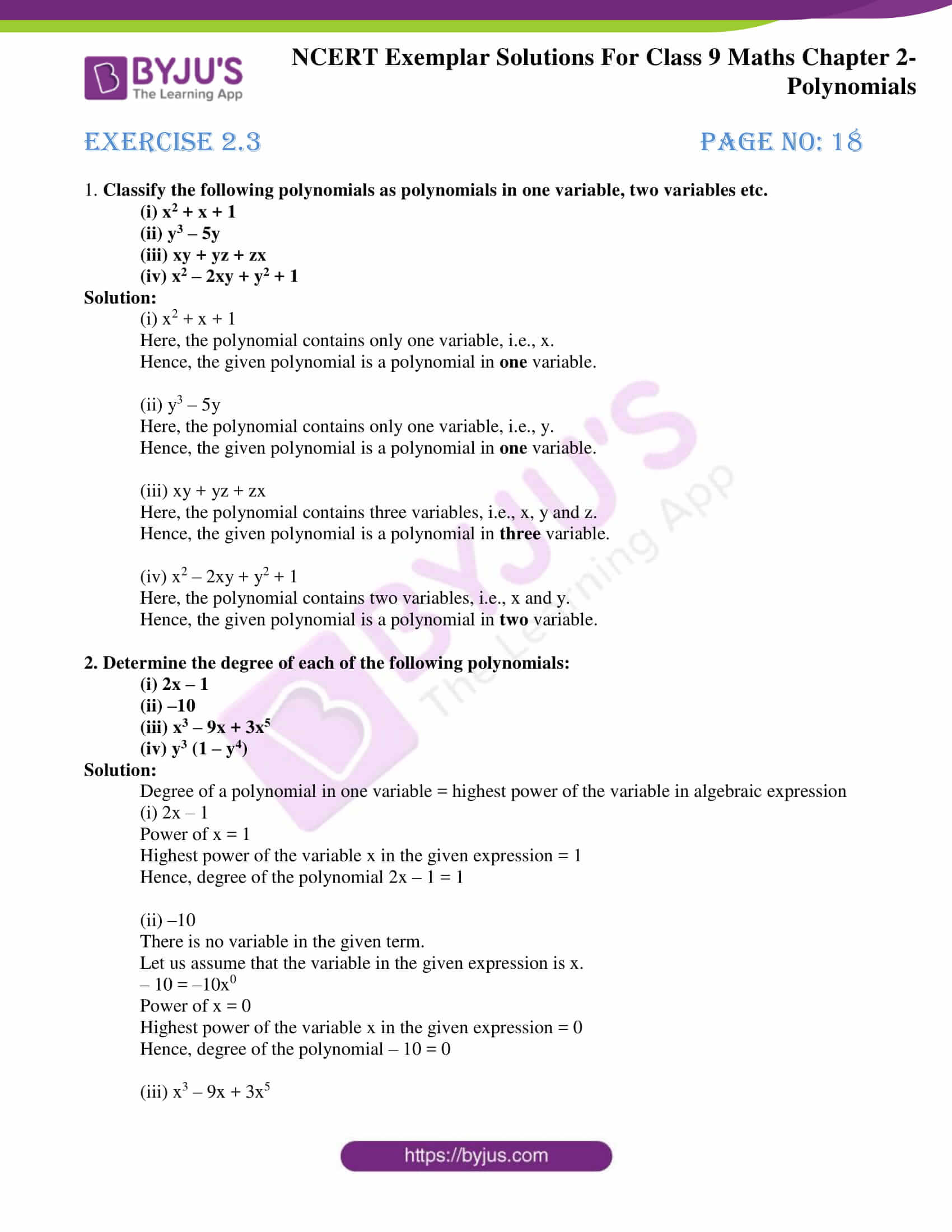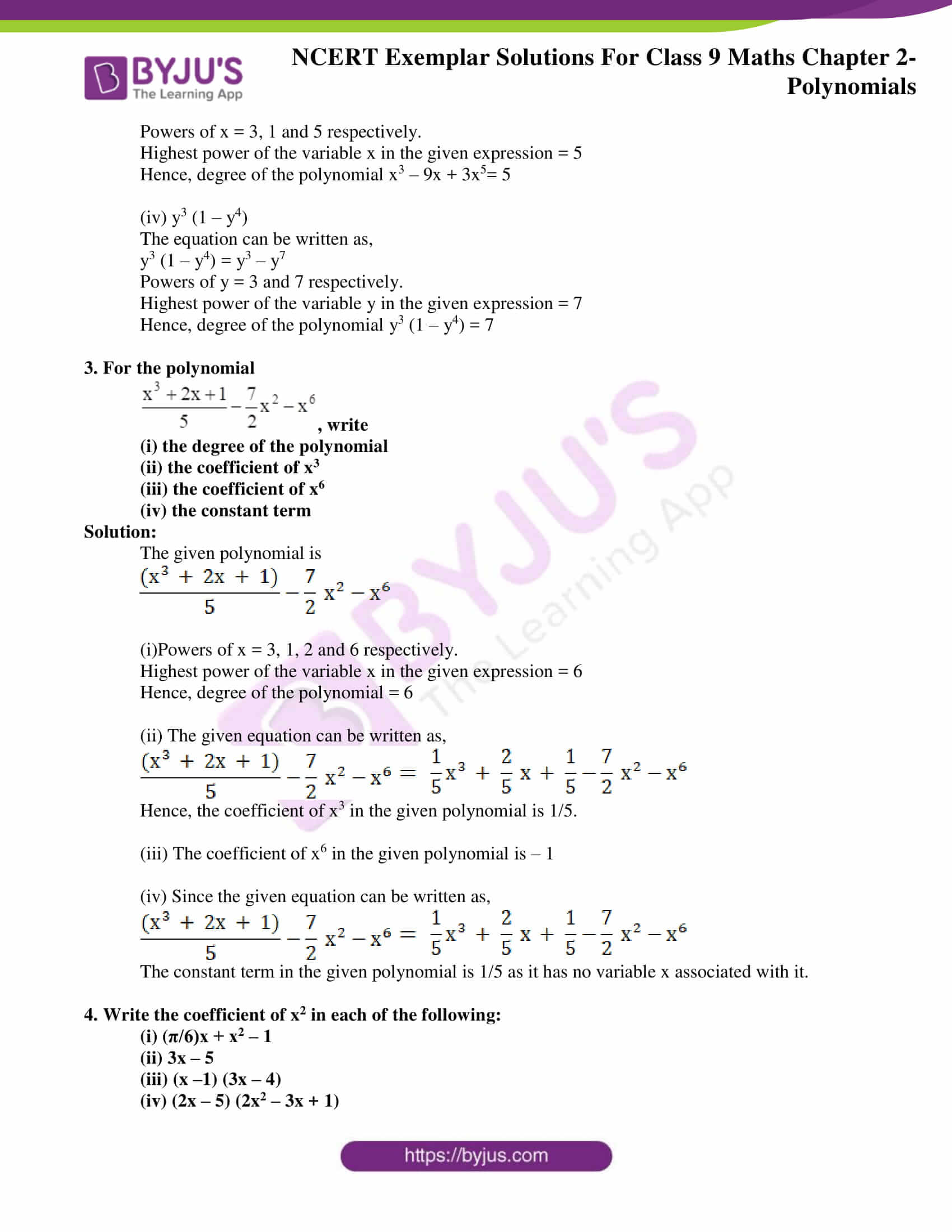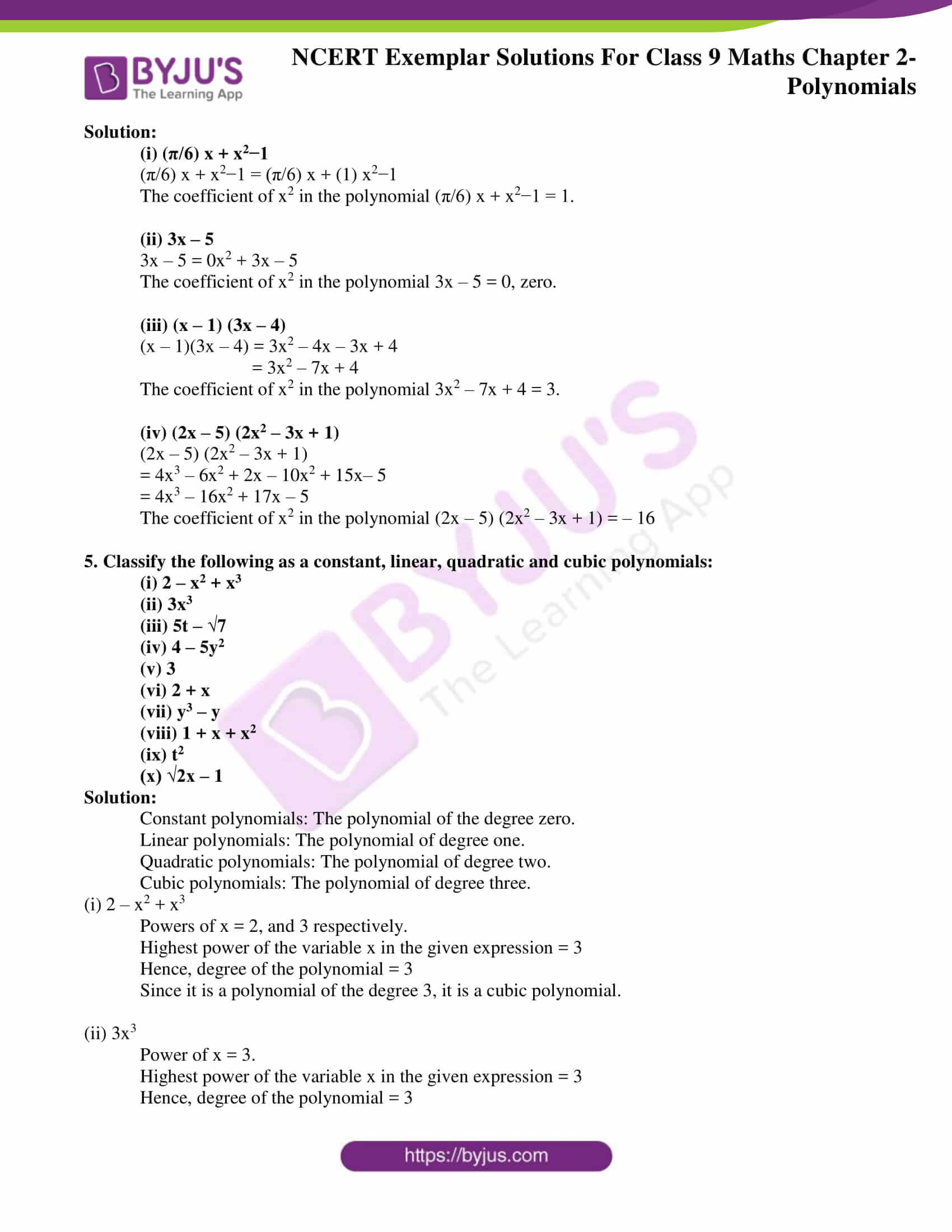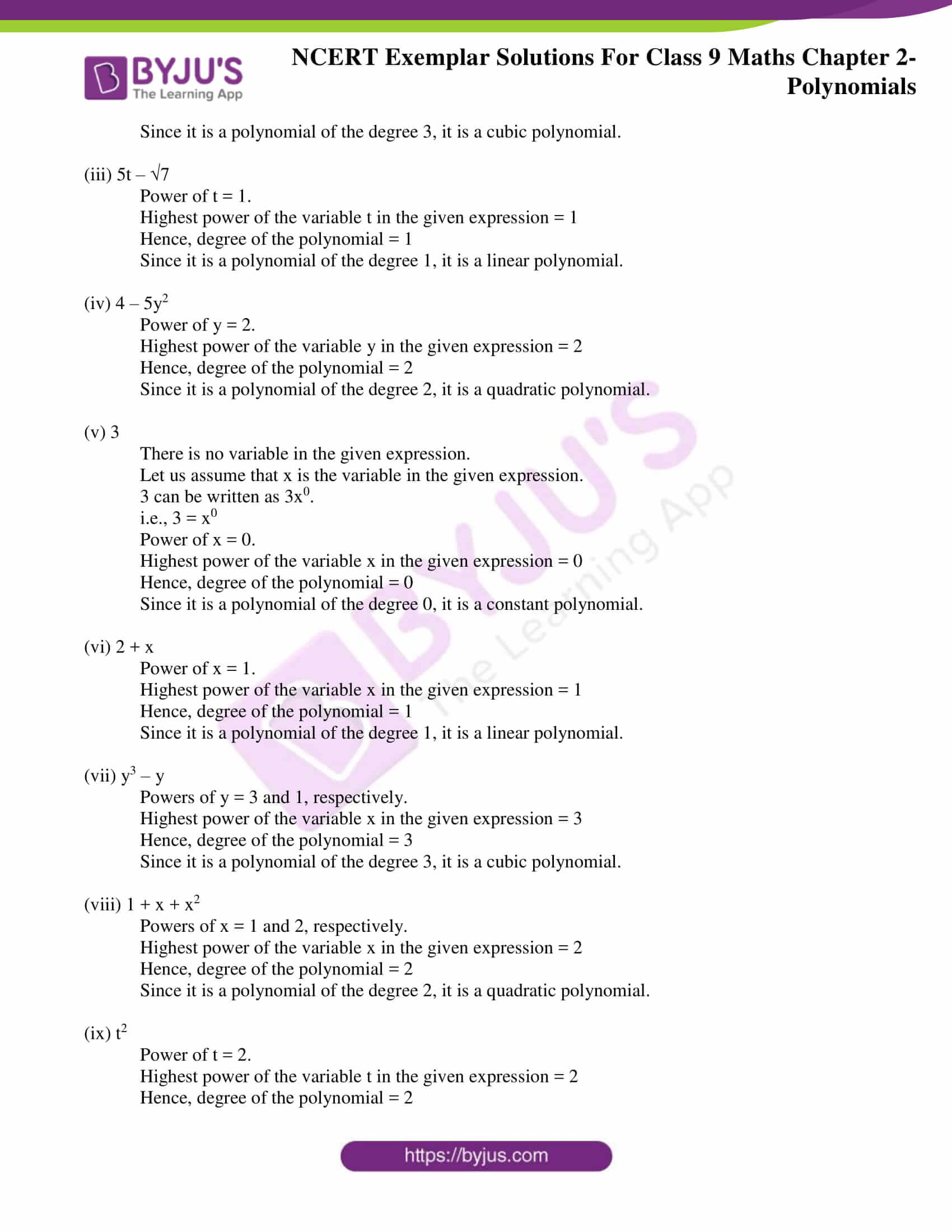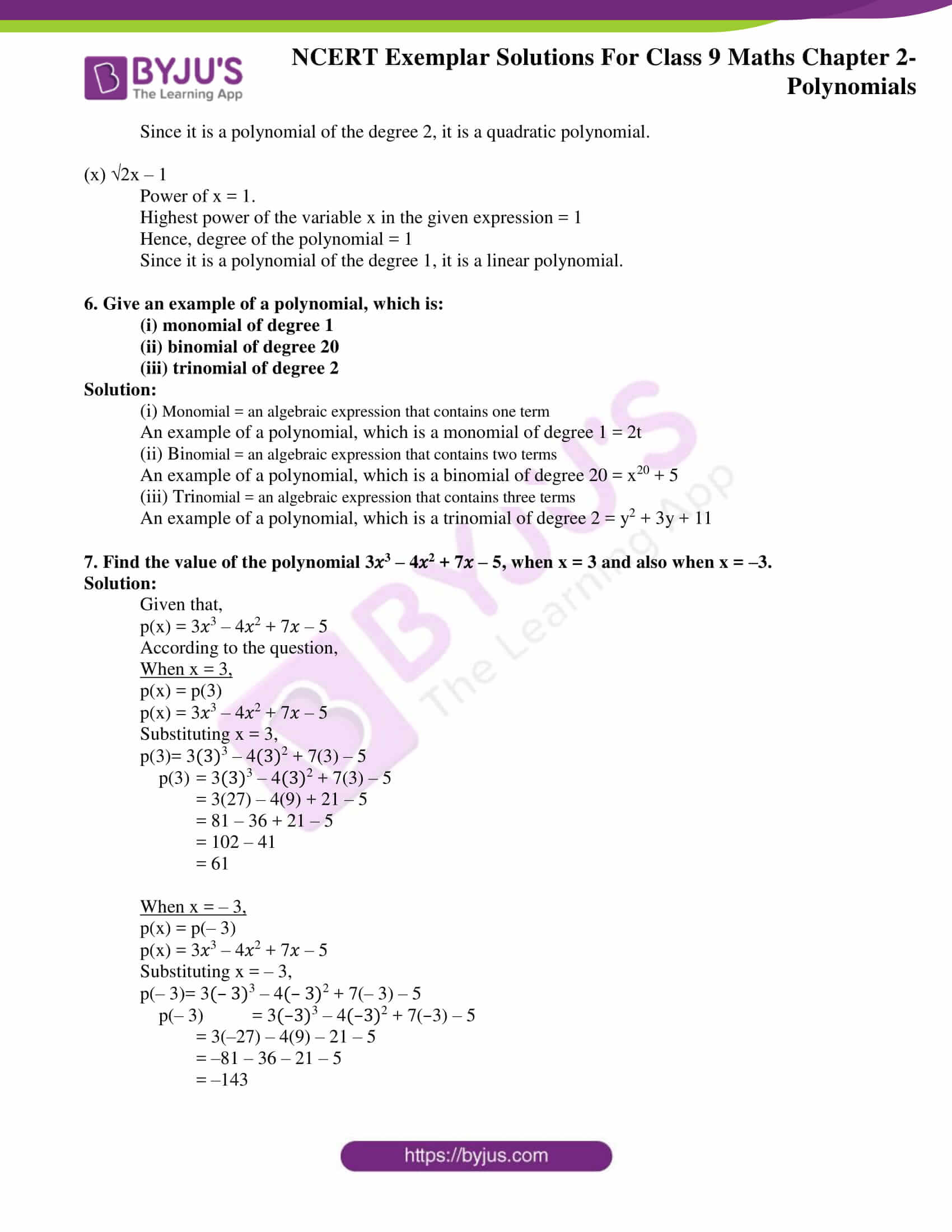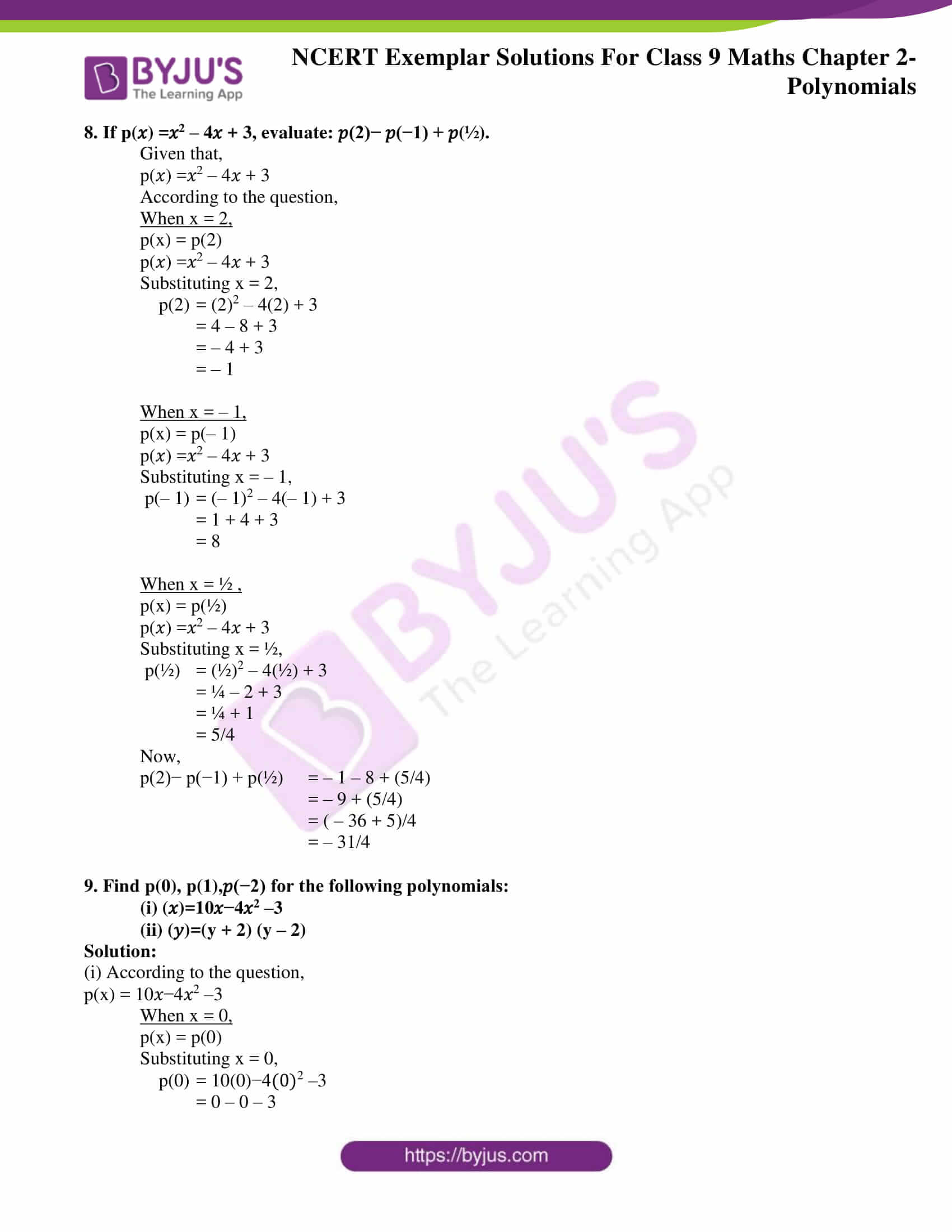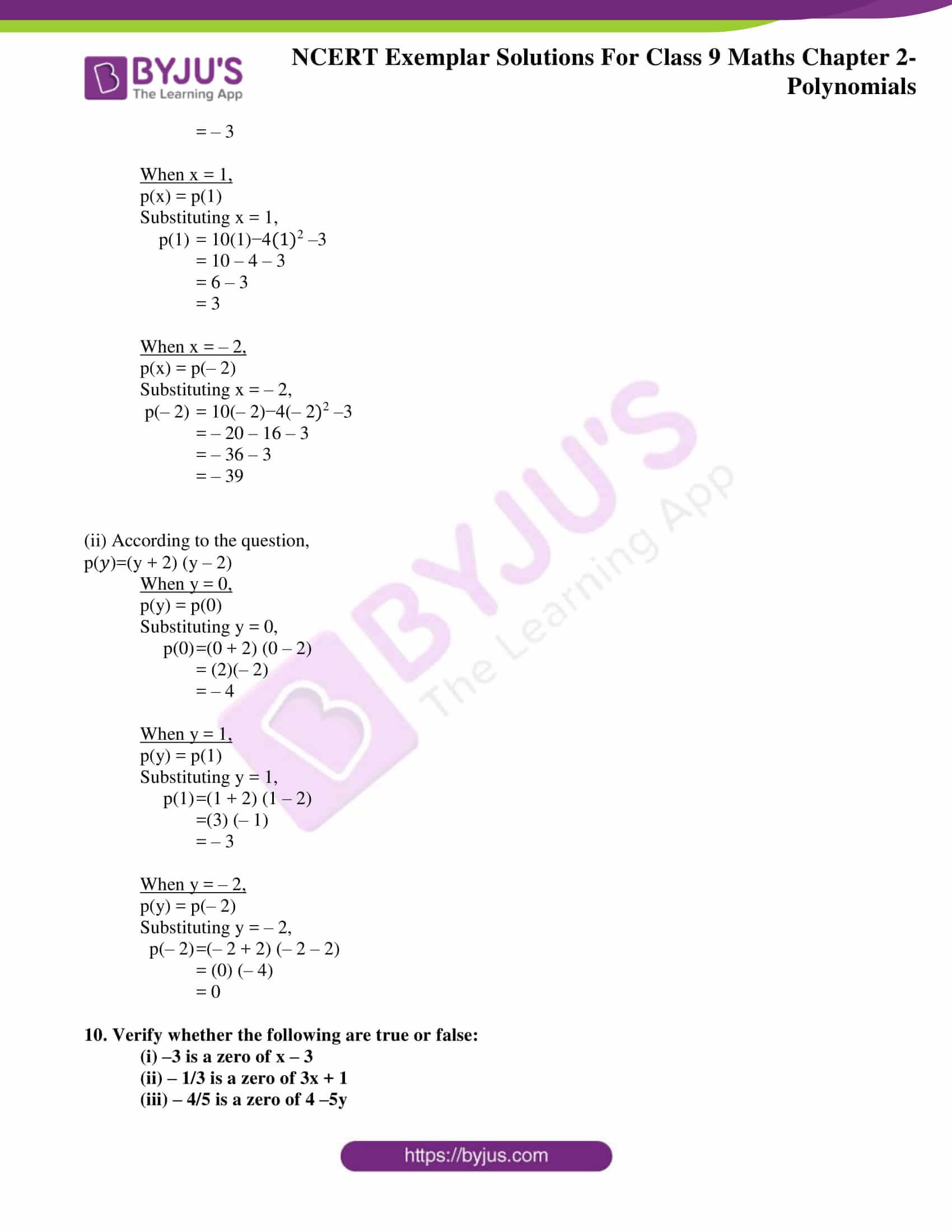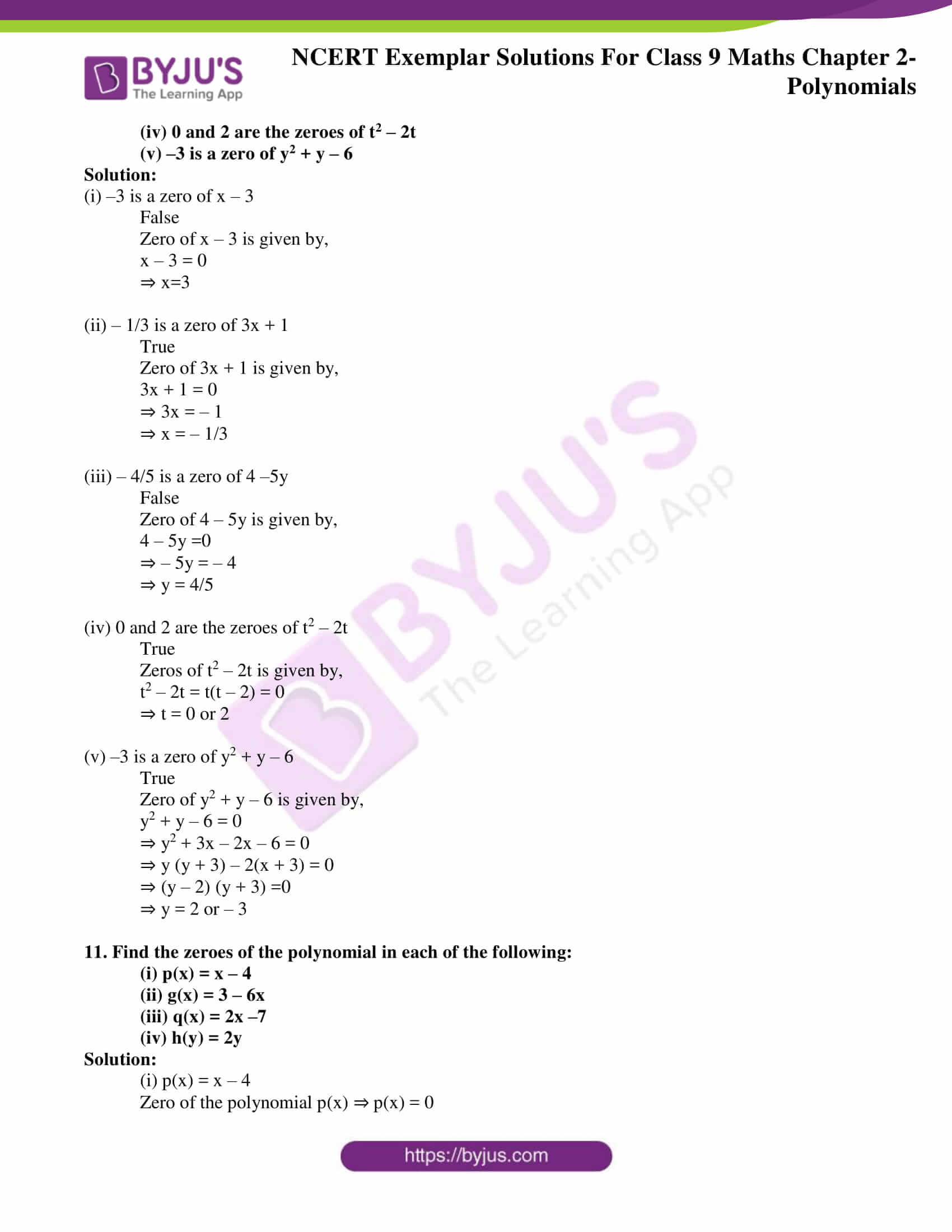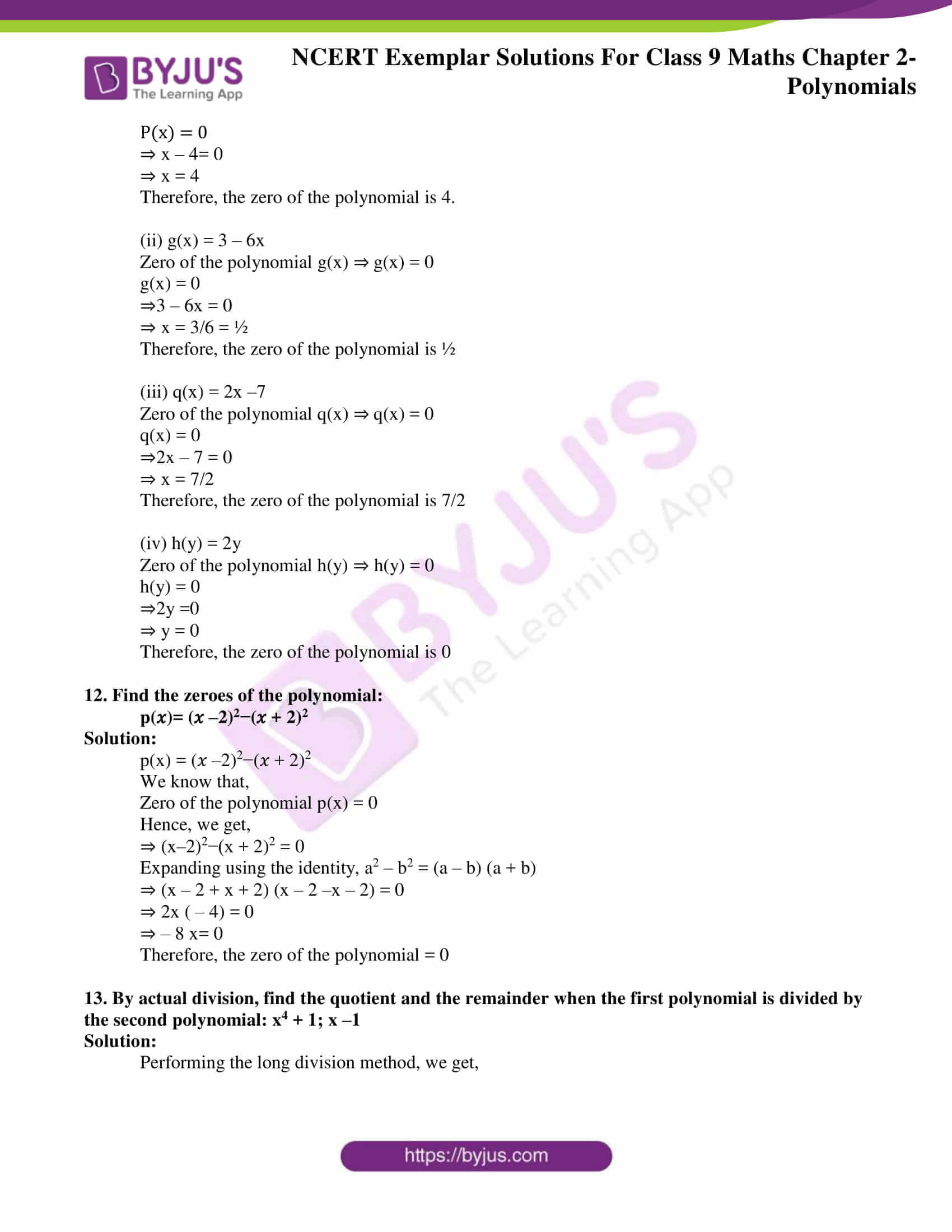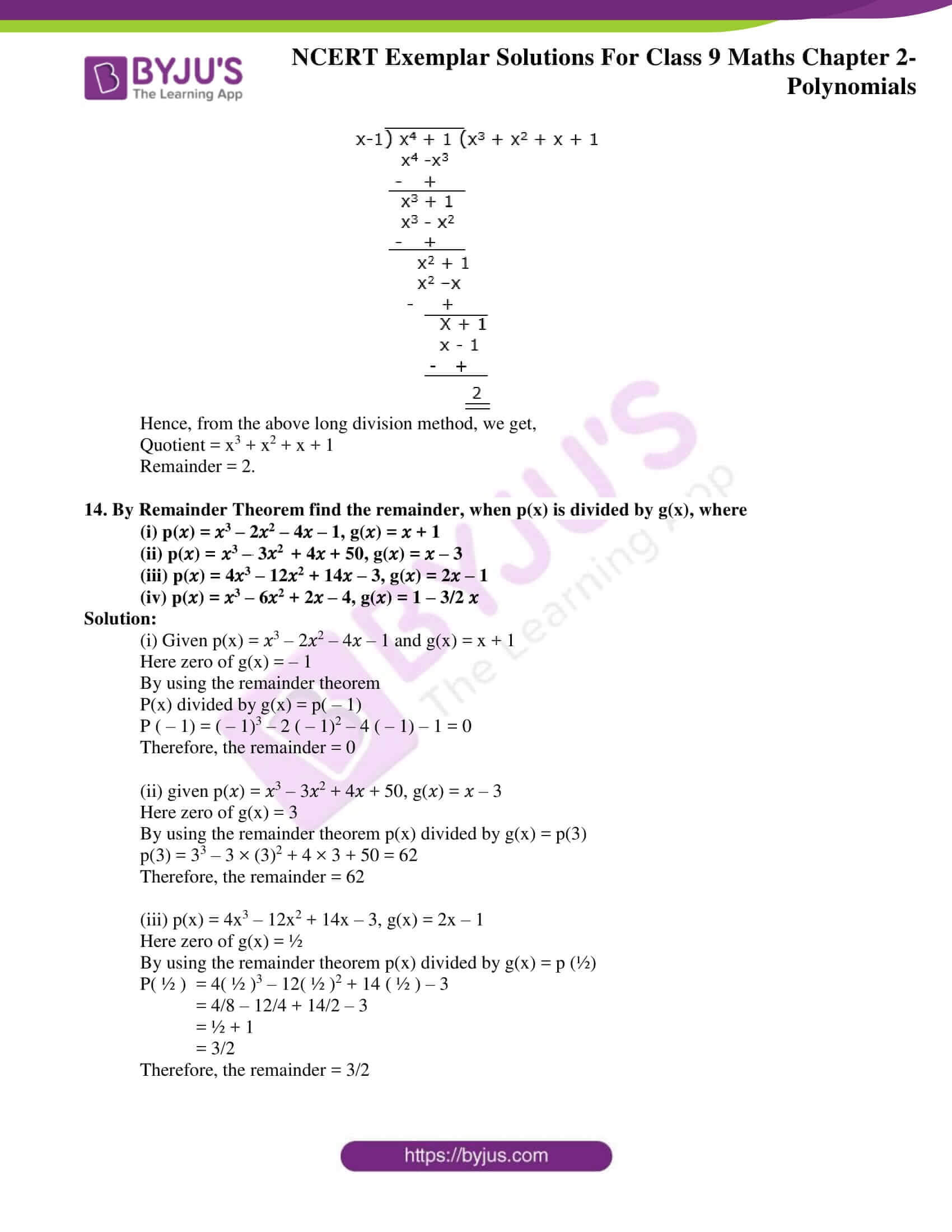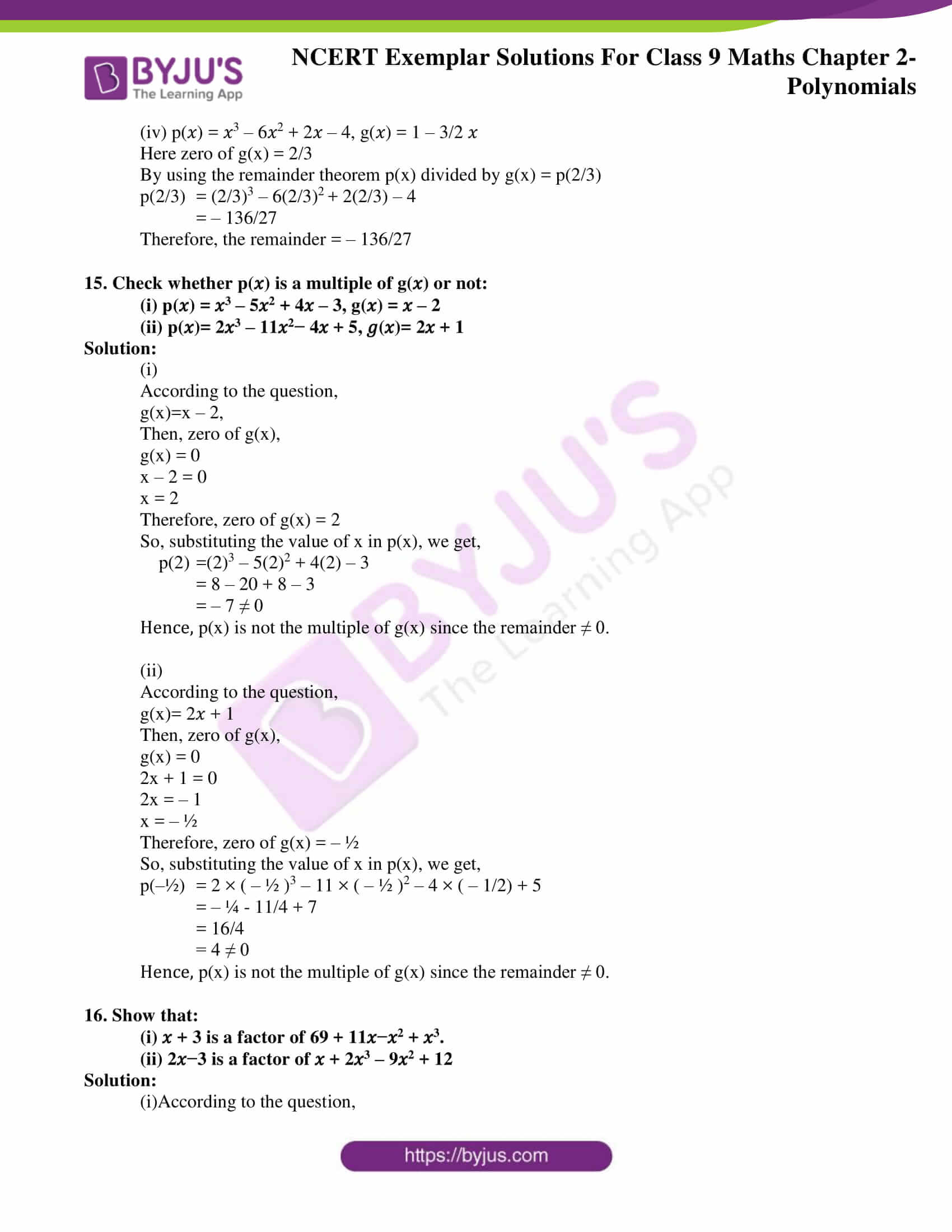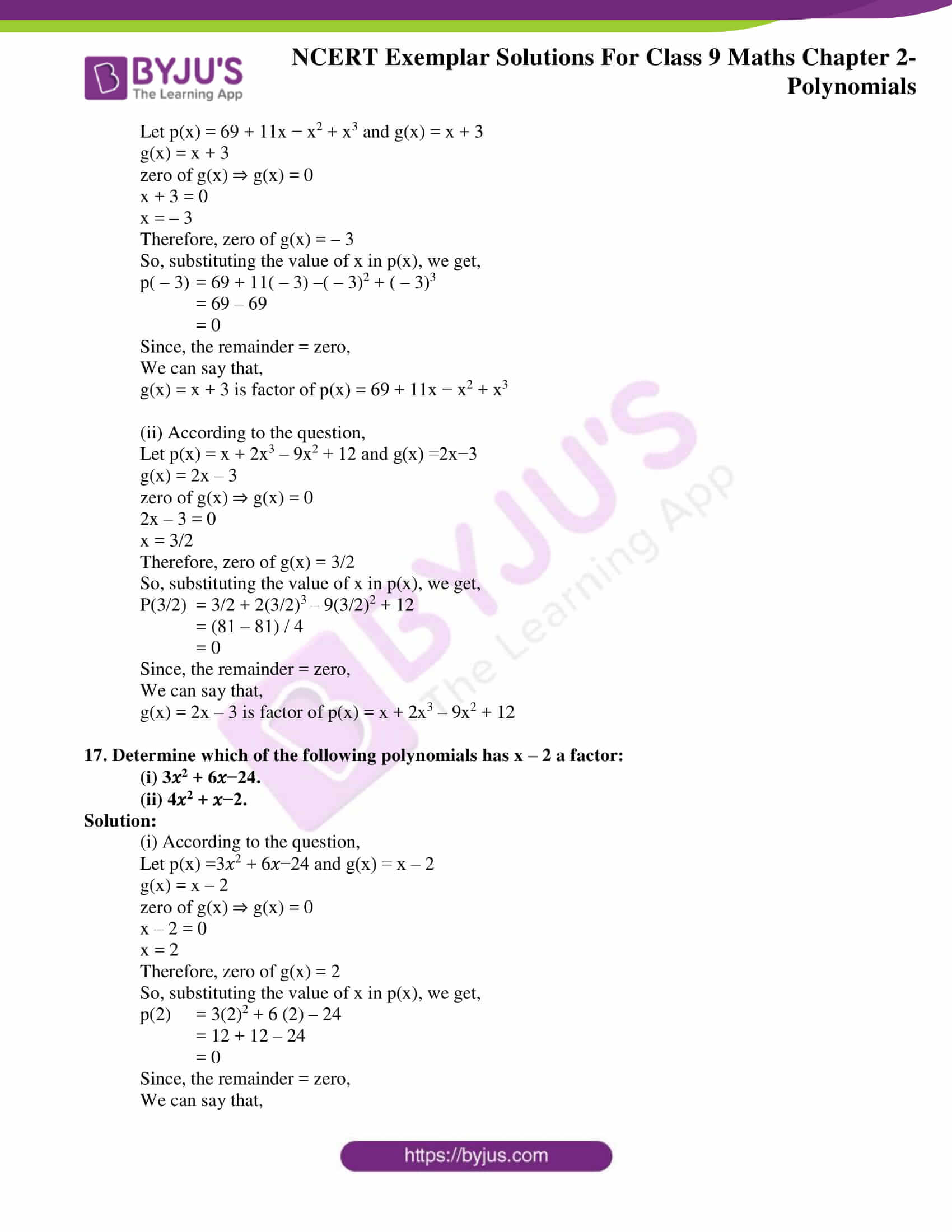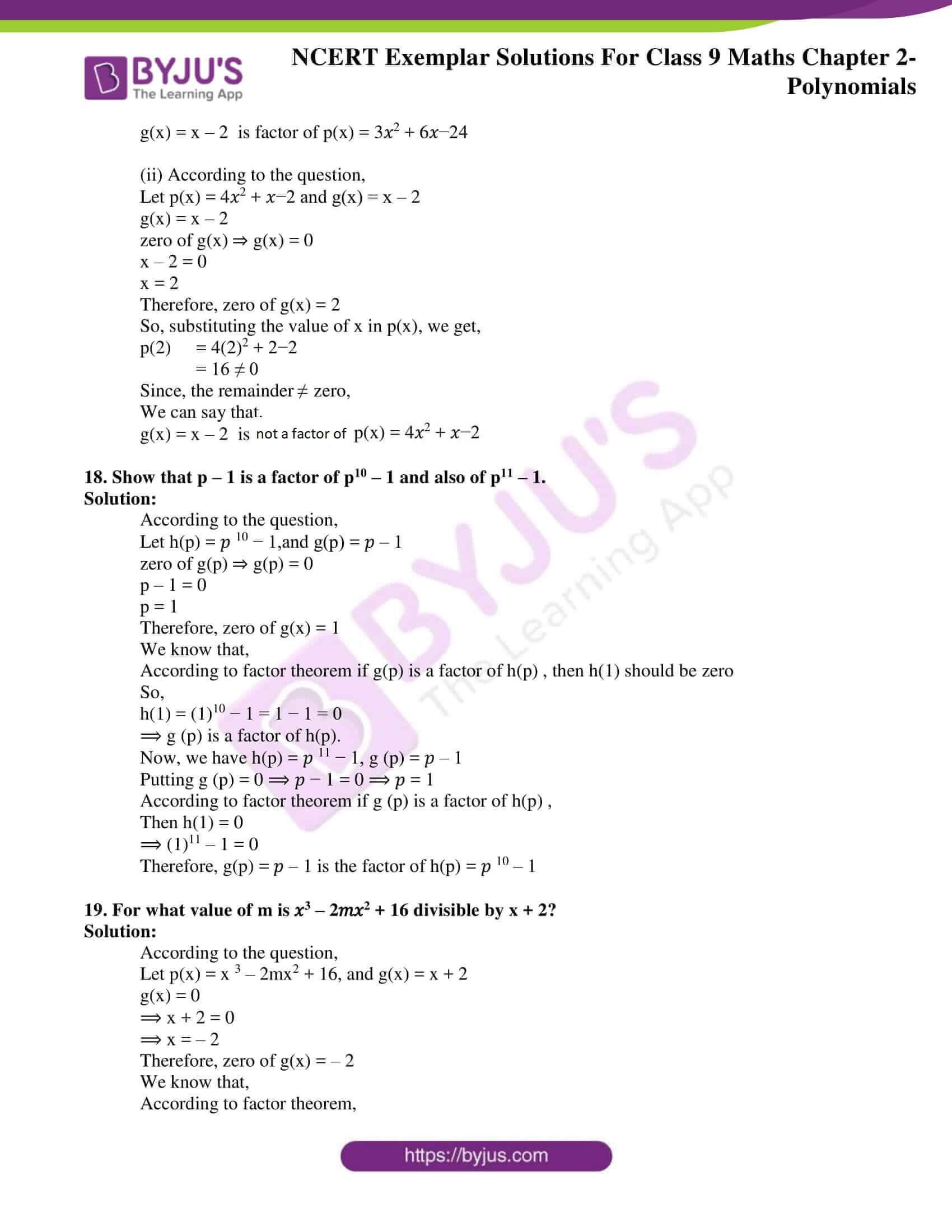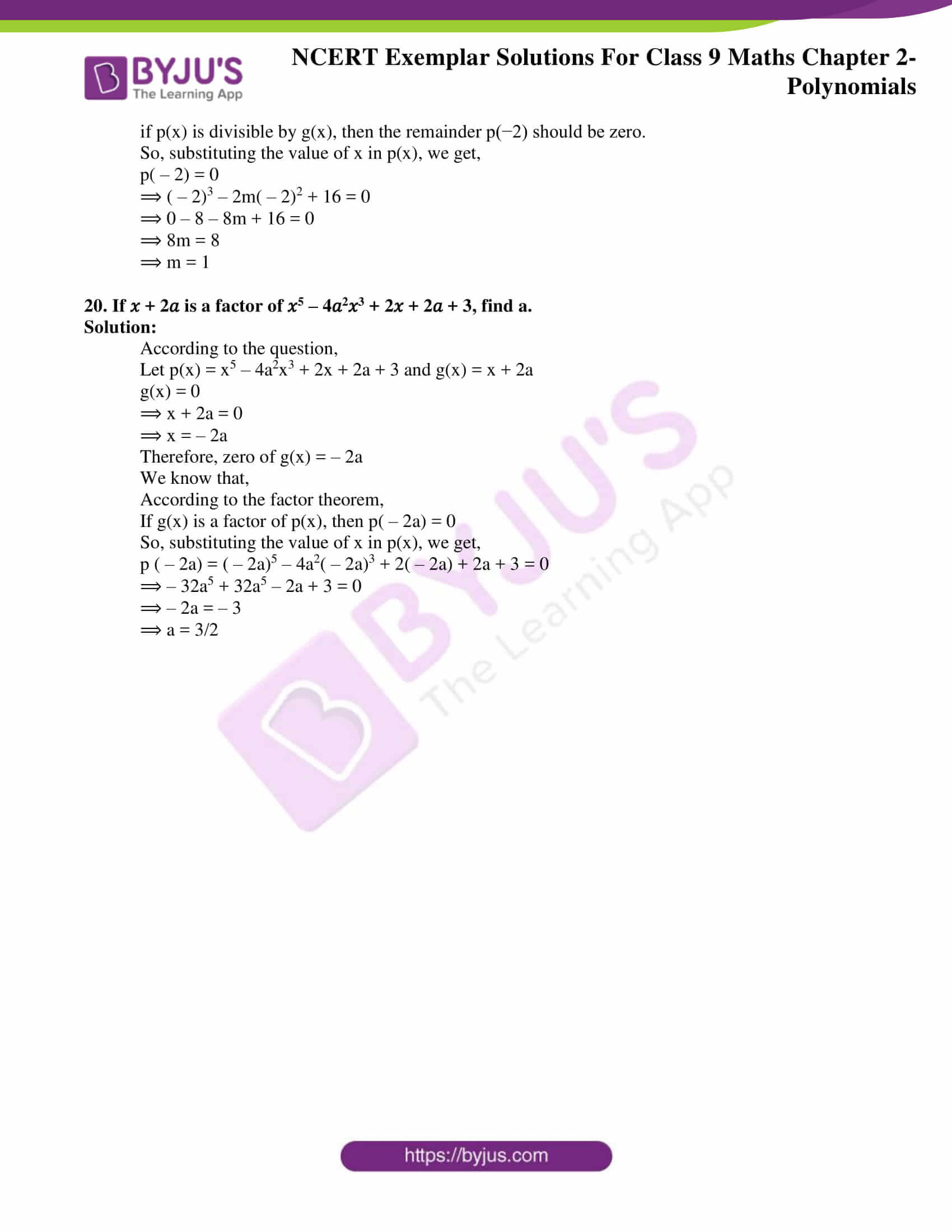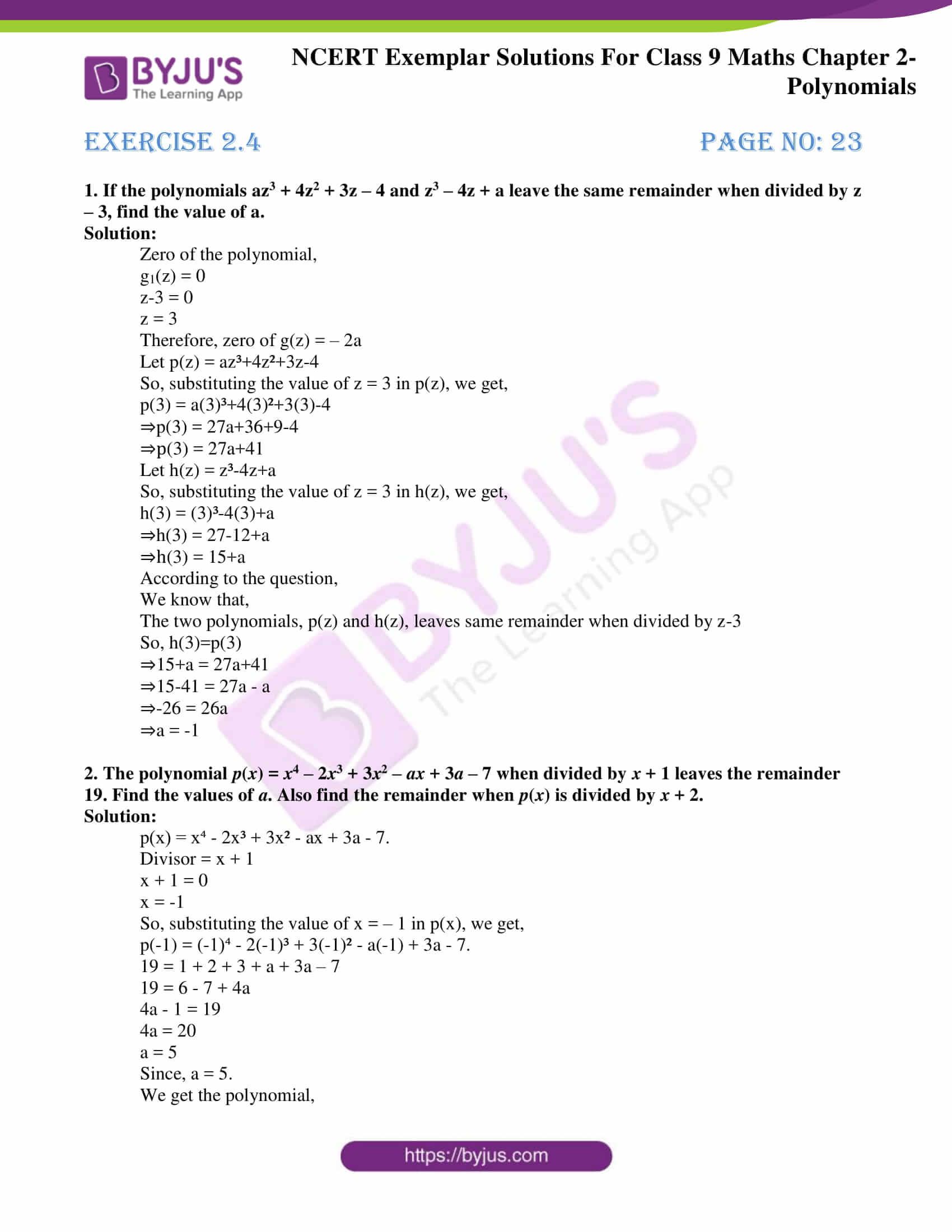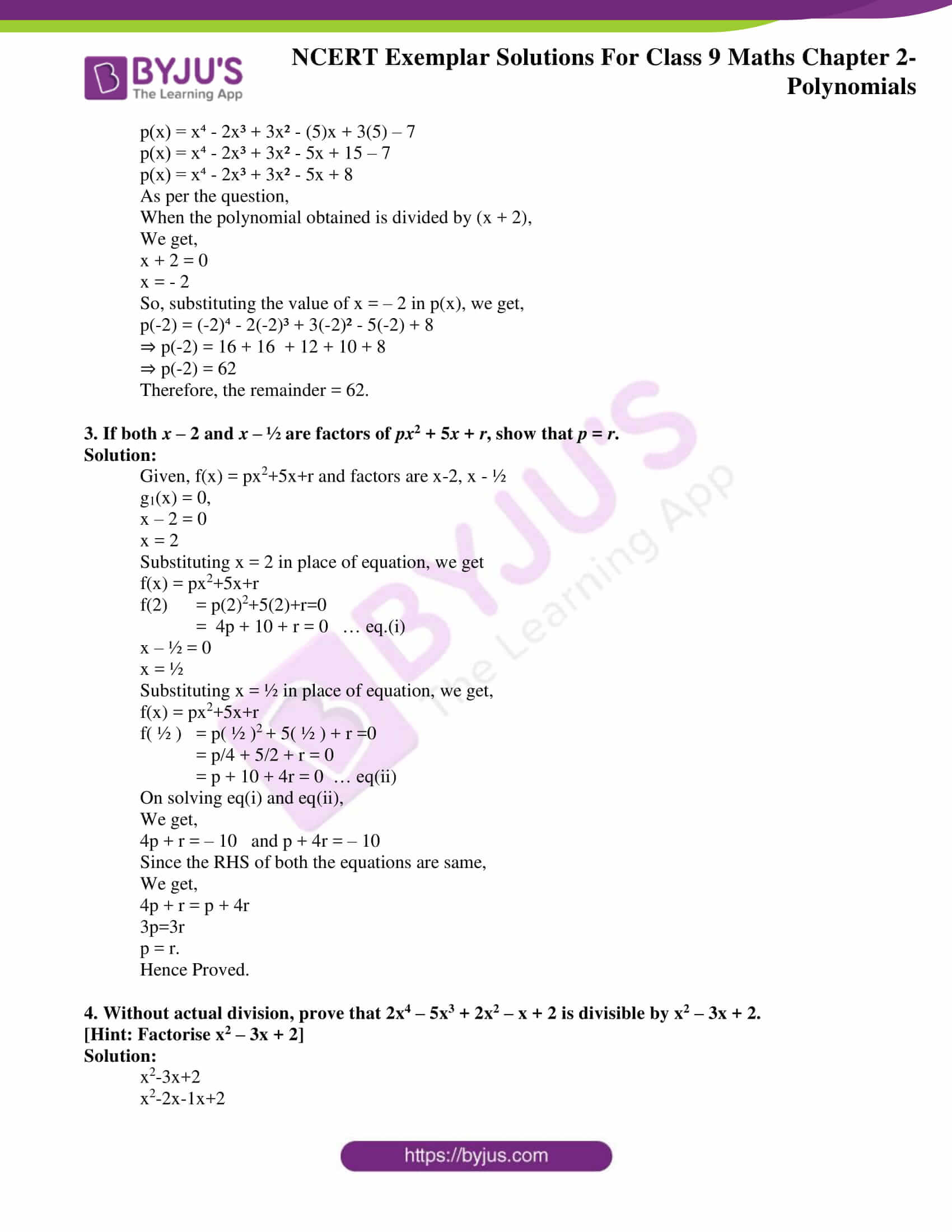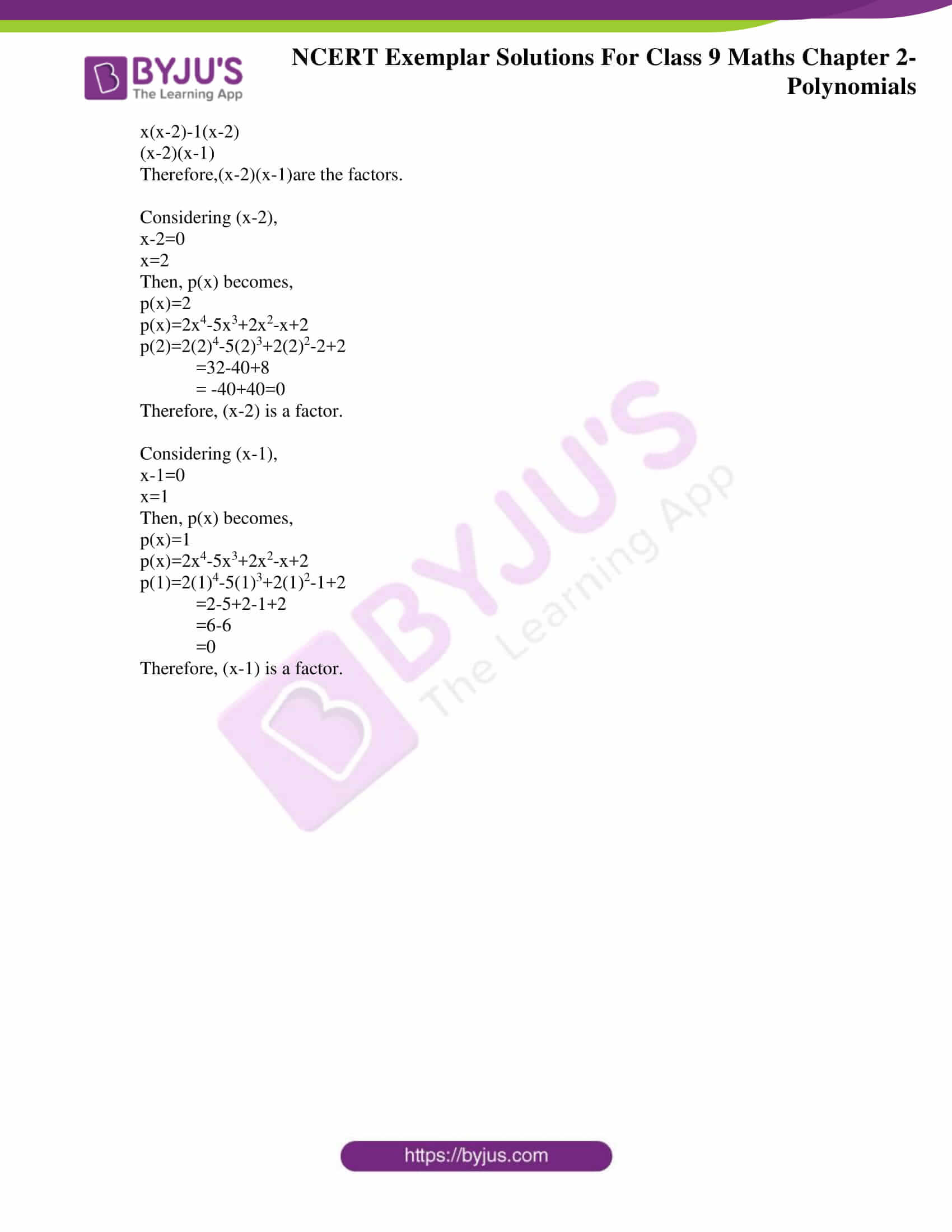### Access answers to NCERT Exemplar Solutions for Class 9 Maths Chapter 2 Polynomials

Exercise 2.1 Page No: 14

Write the correct answer in each of the following:

1. Which one of the following is a polynomial?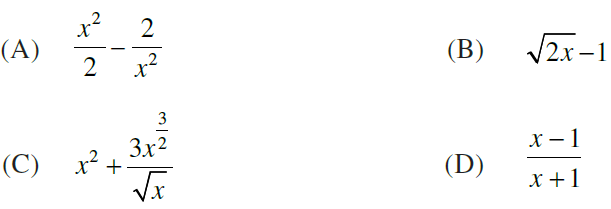Solution:

(C)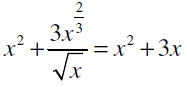Explanation:

(A)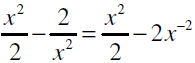The equation contains the term x2 and -2x-2.

Here, the exponent of x in second term = – 2, which is not a whole number.

Hence, the given algebraic expression is not a polynomial.

(B)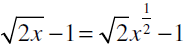The equation contains the term √2x½ .

Here, the exponent of x in first term = ½ , which is not a whole number.

Hence, the given algebraic expression is not a polynomial.

(C)The equation contains the term x2 and 3x .

Here, the exponent of x in first term and second term= 2and 1 respectively, which is a whole number.

Hence, the given algebraic expression is a polynomial.

(D)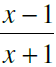The equation is a rational function.

Here, the given equation is not in the standard form of polynomial.

Hence, the given algebraic expression is not a polynomial.

Hence, option C is the correct answer

2. √2 is a polynomial of degree

(A) 2

(B) 0

(C) 1

(D) ½

Solution:

(B) 0

Explanation:

√2 can be written as √2x0

i.e., √2 = √2x0

Therefore, the degree of the polynomial = 0

Hence, option B is the correct answer

3. Degree of the polynomial 4×4 + 0x3 + 0x5 + 5x + 7 is

(A) 4

(B) 5

(C) 3

(D) 7

Solution:

(A) 4

Explanation:

Degree of a polynomial = Highest power of the variable in a polynomial.

The highest power of variable x in the polynomial 4×4 + 0x3 + 0x5 + 5x + 7 is 4.

Therefore, degree of the polynomial of 4×4 + 0x3 + 0x5 + 5x + 7 = 4

Hence, option A is the correct answer

4. Degree of the zero polynomial is

(A) 0

(B) 1

(C) Any natural number

(D) Not defined

Solution:

(D) Not defined

Explanation:

Degree of a zero polynomial is not defined.

Hence, option D is the correct answer

5. If p(x)= x2 – 2√2x + 1, then p(2√2) is equal to

(A) 0

(B) 1

(C) 4√2

(D) 8√2 +1

Solution:

(B) 1

Explanation:

According to the question,

p(x) = x2 – 2√2x + 1

To get p(2√2),

We substitute x = 2√2,

p(2√2) = (2√2)2 – (2√2 × (2√2)) + 1

= (4 × 2) – (4 × 2) + 1

= 8 – 8 + 1

= 1

Hence, option B is the correct answer

6. The value of the polynomial 5x – 4x2 + 3, when x = – 1 is

(A) – 6

(B) 6

(C) 2

(D) – 2

Solution:

(A) – 6

Explanation:

According to the question,

p(x) = 5x – 4x2 + 3

To get p(– 1),

We substitute x = – 1,

p(– 1) = 5(– 1) – 4(– 1)2 + 3

= 5(– 1) – 4(1) + 3

= – 5 – 4 + 3

= – 9 + 3

= – 6

Hence, option A is the correct answer

7. If p(x) = x + 3, then p(x) + p(–x) is equal to

(A) 3

(B) 2x

(C) 0

(D) 6

Solution:

(D) 6

Explanation:

p(x) = x + 3

p(– x) = – x + 3

Therefore,

p(x) + p(–x) = (x + 3) + (– x + 3)

= x + 3 – x + 3

= 6

Hence, option D is the correct answer

8. Zero of the zero polynomial is

(A) 0

(B) 1

(C) Any real number

(D) Not defined

Solution:

(C) Any real number

Explanation:

Zero polynomial is a constant polynomial whose coefficients are all equal to 0.

Zero of a polynomial is the value of the variable that makes the polynomial equal to zero.

Therefore, zero of the zero polynomial is any real number.

Hence, option C is the correct answer

9. Zero of the polynomial p(x) = 2x + 5 is

(A) – 2/5

(B) – 5/2

(C) 2/5

(D) 5/2

Solution:

(B) – 5/2

Explanation:

Zero of the polynomial ⇒ p(x) = 0

p(x) = 0

2x + 5 = 0

2x = – 5

x = – 5/2

Hence, option B is the correct answer

10. One of the zeroes of the polynomial 2x2 + 7x –4 is

(A) 2

(B) ½

(C) – ½

(D) –2

Solution:

(B) ½

Explanation:

Zero of the polynomial ⇒ p(x) = 0

p(x) = 0

2x2 + 7x – 4 = 0

2x2 – 1x + 8x – 4 = 0

x(2x – 1) + 4(2x – 1) = 0

(x + 4)(2x – 1) = 0

Consider, x + 4

x + 4 = 0

x = – 4

Consider, 2x – 1

2x – 1 = 0

2x = 1

x = ½

Hence, option B is the correct answer

Exercise 2.2 Page No: 16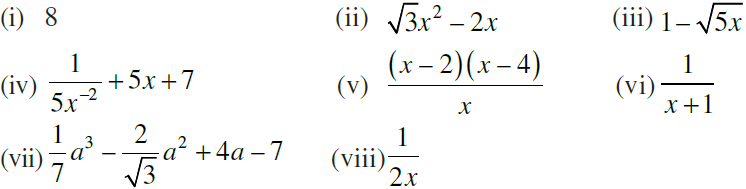Solution:

(i) 8

8 can be written as 8x0.

i.e., 8 = 8x0,

Here, the power of x = 0, which is a whole number.

Hence, 8 is a polynomial.

(ii) √3x2 – 2x

√3x2 – 2x

Here, the power of x are 2 and 1 respectively

2 and 1 both are whole numbers.

Hence, √3x2 – 2x is a polynomial.

(iii) 1 – √(5x)

1 – √5√x = 1 – √5 x½

Here, the power of x = ½, which is not a whole number.

Hence, 1 – √5x is not a polynomial

(iv)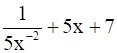1/5x–2 + 5x + 7 = 5x2 + 5x + 7

Here, the power of x are 2 and 1 respectively

2 and 1 both are whole numbers.

Hence, 1/5x-2 + 5x + 7 is a polynomial.

(v)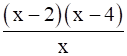((x – 2)(x – 4))/x = (x2 – 4x – 2x + 8)/x

= (x2 – 6x + 8)/x

= x – 6 + (8/x)

= x – 6 + 8x–1

Here, the power of x = – 1, which is not a whole number, but a negative number.

Hence, ((x – 2)(x – 4))/x is not a polynomial

(vi)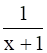1/(x+1) = (x+1)– 1

Here, the power of x is not a whole number.

Hence, 1/(x+1) is not a polynomial

(vii)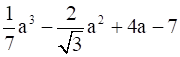(1/7)a3 – (2/√3)a2 + 4a – 7

Here, the power of a are 3, 2 and 1 respectively

3, 2 and 1 are all whole numbers.

Hence, (1/7)a3 – (2/√3)a2 + 4a – 7 is a polynomial.

(viii)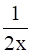1/2x = (x–1/2)

Here, the power of x = – 1, which is not a whole number, but a negative number.

Hence, 1/2x is not a polynomial

Exercise 2.3 Page No: 18

1. Classify the following polynomials as polynomials in one variable, two variables etc.

(i) x2 + x + 1
(ii) y3 – 5y
(iii) xy + yz + zx
(iv) x2 – 2xy + y2 + 1

Solution:

(i) x2 + x + 1

Here, the polynomial contains only one variable, i.e., x.

Hence, the given polynomial is a polynomial in one variable.

(ii) y3 – 5y

Here, the polynomial contains only one variable, i.e., y.

Hence, the given polynomial is a polynomial in one variable.

(iii) xy + yz + zx

Here, the polynomial contains three variables, i.e., x, y and z.

Hence, the given polynomial is a polynomial in three variable.

(iv) x2 – 2xy + y2 + 1

Here, the polynomial contains two variables, i.e., x and y.

Hence, the given polynomial is a polynomial in two variable.

2. Determine the degree of each of the following polynomials:

(i) 2x – 1
(ii) –10
(iii) x3 – 9x + 3x5
(iv) y3 (1 – y4)

Solution:

Degree of a polynomial in one variable = highest power of the variable in algebraic expression

(i) 2x – 1

Power of x = 1

Highest power of the variable x in the given expression = 1

Hence, degree of the polynomial 2x – 1 = 1

(ii) –10

There is no variable in the given term.

Let us assume that the variable in the given expression is x.

– 10 = –10x0

Power of x = 0

Highest power of the variable x in the given expression = 0

Hence, degree of the polynomial – 10 = 0

(iii) x3 – 9x + 3x5

Powers of x = 3, 1 and 5 respectively.

Highest power of the variable x in the given expression = 5

Hence, degree of the polynomial x3 – 9x + 3x5= 5

(iv) y3 (1 – y4)

The equation can be written as,

y3 (1 – y4) = y3 – y7

Powers of y = 3 and 7 respectively.

Highest power of the variable y in the given expression = 7

Hence, degree of the polynomial y3 (1 – y4) = 7

3. For the polynomial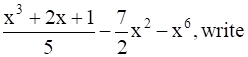, write

(i) the degree of the polynomial
(ii) the coefficient of x3
(iii) the coefficient of x6
(iv) the constant term

Solution:

The given polynomial is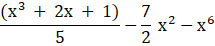(i)Powers of x = 3, 1, 2 and 6 respectively.

Highest power of the variable x in the given expression = 6

Hence, degree of the polynomial = 6

(ii) The given equation can be written as,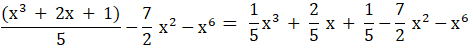Hence, the coefficient of x3 in the given polynomial is 1/5.

(iii) The coefficient of x6 in the given polynomial is – 1

(iv) Since the given equation can be written as,The constant term in the given polynomial is 1/5 as it has no variable x associated with it.

4. Write the coefficient of x2 in each of the following:

(i) (π/6)x + x2 – 1
(ii) 3x – 5
(iii) (x –1) (3x – 4)
(iv) (2x – 5) (2x2 – 3x + 1)

Solution:

(i) (π/6) x + x2−1

(π/6) x + x2−1 = (π/6) x + (1) x2−1

The coefficient of x2 in the polynomial (π/6) x + x2−1 = 1.

(ii) 3x – 5

3x – 5 = 0x2 + 3x – 5

The coefficient of x2 in the polynomial 3x – 5 = 0, zero.

(iii) (x – 1) (3x – 4)

(x – 1)(3x – 4) = 3x2 – 4x – 3x + 4

= 3x2 – 7x + 4

The coefficient of x2 in the polynomial 3x2 – 7x + 4 = 3.

(iv) (2x – 5) (2x2 – 3x + 1)

(2x – 5) (2x2 – 3x + 1)

= 4x3 – 6x2 + 2x – 10x2 + 15x– 5

= 4x3 – 16x2 + 17x – 5

The coefficient of x2 in the polynomial (2x – 5) (2x2 – 3x + 1) = – 16

5. Classify the following as a constant, linear, quadratic and cubic polynomials:

(i) 2 – x2 + x3

(ii) 3x3
(iii) 5t – √7

(iv) 4 – 5y2
(v) 3

(vi) 2 + x
(vii) y3 – y

(viii) 1 + x + x2
(ix) t2

(x) √2x – 1

Solution:

Constant polynomials: The polynomial of the degree zero.

Linear polynomials: The polynomial of degree one.

Quadratic polynomials: The polynomial of degree two.

Cubic polynomials: The polynomial of degree three.

(i) 2 – x2 + x3

Powers of x = 2, and 3 respectively.

Highest power of the variable x in the given expression = 3

Hence, degree of the polynomial = 3

Since it is a polynomial of the degree 3, it is a cubic polynomial.

(ii) 3x3

Power of x = 3.

Highest power of the variable x in the given expression = 3

Hence, degree of the polynomial = 3

Since it is a polynomial of the degree 3, it is a cubic polynomial.

(iii) 5t – √7

Power of t = 1.

Highest power of the variable t in the given expression = 1

Hence, degree of the polynomial = 1

Since it is a polynomial of the degree 1, it is a linear polynomial.

(iv) 4 – 5y2

Power of y = 2.

Highest power of the variable y in the given expression = 2

Hence, degree of the polynomial = 2

Since it is a polynomial of the degree 2, it is a quadratic polynomial.

(v) 3

There is no variable in the given expression.

Let us assume that x is the variable in the given expression.

3 can be written as 3x0.

i.e., 3 = x0

Power of x = 0.

Highest power of the variable x in the given expression = 0

Hence, degree of the polynomial = 0

Since it is a polynomial of the degree 0, it is a constant polynomial.

(vi) 2 + x

Power of x = 1.

Highest power of the variable x in the given expression = 1

Hence, degree of the polynomial = 1

Since it is a polynomial of the degree 1, it is a linear polynomial.

(vii) y3 – y

Powers of y = 3 and 1, respectively.

Highest power of the variable x in the given expression = 3

Hence, degree of the polynomial = 3

Since it is a polynomial of the degree 3, it is a cubic polynomial.

(viii) 1 + x + x2

Powers of x = 1 and 2, respectively.

Highest power of the variable x in the given expression = 2

Hence, degree of the polynomial = 2

Since it is a polynomial of the degree 2, it is a quadratic polynomial.

(ix) t2

Power of t = 2.

Highest power of the variable t in the given expression = 2

Hence, degree of the polynomial = 2

Since it is a polynomial of the degree 2, it is a quadratic polynomial.

(x) √2x – 1

Power of x = 1.

Highest power of the variable x in the given expression = 1

Hence, degree of the polynomial = 1

Since it is a polynomial of the degree 1, it is a linear polynomial.

6. Give an example of a polynomial, which is:

(i) monomial of degree 1
(ii) binomial of degree 20
(iii) trinomial of degree 2

Solution:

(i) Monomial = an algebraic expression that contains one term

An example of a polynomial, which is a monomial of degree 1 = 2t

(ii) Binomial = an algebraic expression that contains two terms

An example of a polynomial, which is a binomial of degree 20 = x20 + 5

(iii) Trinomial = an algebraic expression that contains three terms

An example of a polynomial, which is a trinomial of degree 2 = y2 + 3y + 11

7. Find the value of the polynomial 3𝑥3 – 4𝑥2 + 7𝑥 – 5, when x = 3 and also when x = –3.

Solution:

Given that,

p(x) = 3𝑥3 – 4𝑥2 + 7𝑥 – 5

According to the question,

When x = 3,

p(x) = p(3)

p(x) = 3𝑥3 – 4𝑥2 + 7𝑥 – 5

Substituting x = 3,

p(3)= 3(3)3 – 4(3)2 + 7(3) – 5

p(3) = 3(3)3 – 4(3)2 + 7(3) – 5

= 3(27) – 4(9) + 21 – 5

= 81 – 36 + 21 – 5

= 102 – 41

= 61

When x = – 3,

p(x) = p(– 3)

p(x) = 3𝑥3 – 4𝑥2 + 7𝑥 – 5

Substituting x = – 3,

p(– 3)= 3(– 3)3 – 4(– 3)2 + 7(– 3) – 5

p(– 3) = 3(–3)3 – 4(–3)2 + 7(–3) – 5

= 3(–27) – 4(9) – 21 – 5

= –81 – 36 – 21 – 5

= –143

8. If p(𝑥) =𝑥2 – 4𝑥 + 3, evaluate: 𝑝(2)− 𝑝(−1) + 𝑝(½).

Given that,

p(𝑥) =𝑥2 – 4𝑥 + 3

According to the question,

When x = 2,

p(x) = p(2)

p(𝑥) =𝑥2 – 4𝑥 + 3

Substituting x = 2,

p(2) = (2)2 – 4(2) + 3

= 4 – 8 + 3

= – 4 + 3

= – 1

When x = – 1,

p(x) = p(– 1)

p(𝑥) =𝑥2 – 4𝑥 + 3

Substituting x = – 1,

p(– 1) = (– 1)2 – 4(– 1) + 3

= 1 + 4 + 3

= 8

When x = ½ ,

p(x) = p(½)

p(𝑥) =𝑥2 – 4𝑥 + 3

Substituting x = ½,

p(½) = (½)2 – 4(½) + 3

= ¼ – 2 + 3

= ¼ + 1

= 5/4

Now,

p(2)− p(−1) + p(½) = – 1 – 8 + (5/4)

= – 9 + (5/4)

= ( – 36 + 5)/4

= – 31/4

9. Find p(0), p(1),𝑝(−2) for the following polynomials:

(i) (𝑥)=10𝑥−4𝑥2 –3

(ii) (𝑦)=(y + 2) (y – 2)

Solution:

(i) According to the question,

p(x) = 10𝑥−4𝑥2 –3

When x = 0,

p(x) = p(0)

Substituting x = 0,

p(0) = 10(0)−4(0)2 –3

= 0 – 0 – 3

= – 3

When x = 1,

p(x) = p(1)

Substituting x = 1,

p(1) = 10(1)−4(1)2 –3

= 10 – 4 – 3

= 6 – 3

= 3

When x = – 2,

p(x) = p(– 2)

Substituting x = – 2,

p(– 2) = 10(– 2)−4(– 2)2 –3

= – 20 – 16 – 3

= – 36 – 3

= – 39

(ii) According to the question,

p(𝑦)=(y + 2) (y – 2)

When y = 0,

p(y) = p(0)

Substituting y = 0,

p(0) =(0 + 2) (0 – 2)

= (2)(– 2)

= – 4

When y = 1,

p(y) = p(1)

Substituting y = 1,

p(1) =(1 + 2) (1 – 2)

=(3) (– 1)

= – 3

When y = – 2,

p(y) = p(– 2)

Substituting y = – 2,

p(– 2) =(– 2 + 2) (– 2 – 2)

= (0) (– 4)

= 0

10. Verify whether the following are true or false:

(i) –3 is a zero of x – 3
(ii) – 1/3 is a zero of 3x + 1
(iii) – 4/5 is a zero of 4 –5y
(iv) 0 and 2 are the zeroes of t2 – 2t
(v) –3 is a zero of y2 + y – 6

Solution:

(i) –3 is a zero of x – 3

False

Zero of x – 3 is given by,

x – 3 = 0

⇒ x=3

(ii) – 1/3 is a zero of 3x + 1

True

Zero of 3x + 1 is given by,

3x + 1 = 0

⇒ 3x = – 1

⇒ x = – 1/3

(iii) – 4/5 is a zero of 4 –5y

False

Zero of 4 – 5y is given by,

4 – 5y =0

⇒ – 5y = – 4

⇒ y = 4/5

(iv) 0 and 2 are the zeroes of t2 – 2t

True

Zeros of t2 – 2t is given by,

t2 – 2t = t(t – 2) = 0

⇒ t = 0 or 2

(v) –3 is a zero of y2 + y – 6

True

Zero of y2 + y – 6 is given by,

y2 + y – 6 = 0

⇒ y2 + 3x – 2x – 6 = 0

⇒ y (y + 3) – 2(x + 3) = 0

⇒ (y – 2) (y + 3) =0

⇒ y = 2 or – 3

11. Find the zeroes of the polynomial in each of the following:

(i) p(x) = x – 4
(ii) g(x) = 3 – 6x
(iii) q(x) = 2x –7
(iv) h(y) = 2y

Solution:

(i) p(x) = x – 4

Zero of the polynomial p(x) ⇒ p(x) = 0

P(x) = 0

⇒ x – 4= 0

⇒ x = 4

Therefore, the zero of the polynomial is 4.

(ii) g(x) = 3 – 6x

Zero of the polynomial g(x) ⇒ g(x) = 0

g(x) = 0

⇒3 – 6x = 0

⇒ x = 3/6 = ½

Therefore, the zero of the polynomial is ½

(iii) q(x) = 2x –7

Zero of the polynomial q(x) ⇒ q(x) = 0

q(x) = 0

⇒2x – 7 = 0

⇒ x = 7/2

Therefore, the zero of the polynomial is 7/2

(iv) h(y) = 2y

Zero of the polynomial h(y) ⇒ h(y) = 0

h(y) = 0

⇒2y =0

⇒ y = 0

Therefore, the zero of the polynomial is 0

12. Find the zeroes of the polynomial:

p(𝑥)= (𝑥 –2)2−(𝑥 + 2)2

Solution:

p(x) = (𝑥 –2)2−(𝑥 + 2)2

We know that,

Zero of the polynomial p(x) = 0

Hence, we get,

⇒ (x–2)2−(x + 2)2 = 0

Expanding using the identity, a2 – b2 = (a – b) (a + b)

⇒ (x – 2 + x + 2) (x – 2 –x – 2) = 0

⇒ 2x ( – 4) = 0

⇒ – 8 x= 0

Therefore, the zero of the polynomial = 0

13. By actual division, find the quotient and the remainder when the first polynomial is divided by the second polynomial: x4 + 1; x –1

Solution:

Performing the long division method, we get,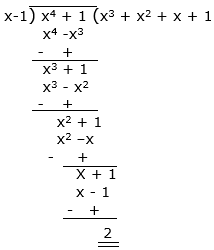Hence, from the above long division method, we get,

Quotient = x3 + x2 + x + 1

Remainder = 2.

14. By Remainder Theorem find the remainder, when p(x) is divided by g(x), where

(i) p(𝑥) = 𝑥3 – 2𝑥2 – 4𝑥 – 1, g(𝑥) = 𝑥 + 1
(ii) p(𝑥) = 𝑥3 – 3𝑥2 + 4𝑥 + 50, g(𝑥) = 𝑥 – 3
(iii) p(𝑥) = 4𝑥3 – 12𝑥2 + 14𝑥 – 3, g(𝑥) = 2𝑥 – 1
(iv) p(𝑥) = 𝑥3 – 6𝑥2 + 2𝑥 – 4, g(𝑥) = 1 – 3/2 𝑥

Solution:

(i) Given p(x) = 𝑥3 – 2𝑥2 – 4𝑥 – 1 and g(x) = x + 1

Here zero of g(x) = – 1

By using the remainder theorem

P(x) divided by g(x) = p( – 1)

P ( – 1) = ( – 1)3 – 2 ( – 1)2 – 4 ( – 1) – 1 = 0

Therefore, the remainder = 0

(ii) given p(𝑥) = 𝑥3 – 3𝑥2 + 4𝑥 + 50, g(𝑥) = 𝑥 – 3

Here zero of g(x) = 3

By using the remainder theorem p(x) divided by g(x) = p(3)

p(3) = 33 – 3 × (3)2 + 4 × 3 + 50 = 62

Therefore, the remainder = 62

(iii) p(x) = 4x3 – 12x2 + 14x – 3, g(x) = 2x – 1

Here zero of g(x) = ½

By using the remainder theorem p(x) divided by g(x) = p (½)

P( ½ ) = 4( ½ )3 – 12( ½ )2 + 14 ( ½ ) – 3

= 4/8 – 12/4 + 14/2 – 3

= ½ + 1

= 3/2

Therefore, the remainder = 3/2

(iv) p(𝑥) = 𝑥3 – 6𝑥2 + 2𝑥 – 4, g(𝑥) = 1 – 3/2 𝑥

Here zero of g(x) = 2/3

By using the remainder theorem p(x) divided by g(x) = p(2/3)

p(2/3) = (2/3)3 – 6(2/3)2 + 2(2/3) – 4

= – 136/27

Therefore, the remainder = – 136/27

15. Check whether p(𝑥) is a multiple of g(𝑥) or not:

(i) p(𝑥) = 𝑥3 – 5𝑥2 + 4𝑥 – 3, g(𝑥) = 𝑥 – 2
(ii) p(𝑥)= 2𝑥3 – 11𝑥2− 4𝑥 + 5, 𝑔(𝑥)= 2𝑥 + 1

Solution:

(i)

According to the question,

g(x)=x – 2,

Then, zero of g(x),

g(x) = 0

x – 2 = 0

x = 2

Therefore, zero of g(x) = 2

So, substituting the value of x in p(x), we get,

p(2) =(2)3 – 5(2)2 + 4(2) – 3

= 8 – 20 + 8 – 3

= – 7 ≠ 0

Hence, p(x) is not the multiple of g(x) since the remainder ≠ 0.

(ii)

According to the question,

g(x)= 2𝑥 + 1

Then, zero of g(x),

g(x) = 0

2x + 1 = 0

2x = – 1

x = – ½

Therefore, zero of g(x) = – ½

So, substituting the value of x in p(x), we get,

p(–½) = 2 × ( – ½ )3 – 11 × ( – ½ )2 – 4 × ( – 1/2) + 5

= – ¼ – 11/4 + 7

= 16/4

= 4 ≠ 0

Hence, p(x) is not the multiple of g(x) since the remainder ≠ 0.

16. Show that:

(i) 𝑥 + 3 is a factor of 69 + 11𝑥−𝑥2 + 𝑥3.
(ii) 2𝑥−3 is a factor of 𝑥 + 2𝑥3 – 9𝑥2 + 12

Solution:

(i)According to the question,

Let p(x) = 69 + 11x − x2 + x3 and g(x) = x + 3

g(x) = x + 3

zero of g(x) ⇒ g(x) = 0

x + 3 = 0

x = – 3

Therefore, zero of g(x) = – 3

So, substituting the value of x in p(x), we get,

p( – 3) = 69 + 11( – 3) –( – 3)2 + ( – 3)3

= 69 – 69

= 0

Since, the remainder = zero,

We can say that,

g(x) = x + 3 is factor of p(x) = 69 + 11x − x2 + x3

(ii) According to the question,

Let p(x) = x + 2x3 – 9x2 + 12 and g(x) =2x−3

g(x) = 2x – 3

zero of g(x) ⇒ g(x) = 0

2x – 3 = 0

x = 3/2

Therefore, zero of g(x) = 3/2

So, substituting the value of x in p(x), we get,

P(3/2) = 3/2 + 2(3/2)3 – 9(3/2)2 + 12

= (81 – 81) / 4

= 0

Since, the remainder = zero,

We can say that,

g(x) = 2x – 3 is factor of p(x) = x + 2x3 – 9x2 + 12

17. Determine which of the following polynomials has x – 2 a factor:

(i) 3𝑥2 + 6𝑥−24.
(ii) 4𝑥2 + 𝑥−2.

Solution:

(i) According to the question,

Let p(x) =3𝑥2 + 6𝑥−24 and g(x) = x – 2

g(x) = x – 2

zero of g(x) ⇒ g(x) = 0

x – 2 = 0

x = 2

Therefore, zero of g(x) = 2

So, substituting the value of x in p(x), we get,

p(2) = 3(2)2 + 6 (2) – 24

= 12 + 12 – 24

= 0

Since, the remainder = zero,

We can say that,

g(x) = x – 2 is factor of p(x) = 3𝑥2 + 6𝑥−24

(ii) According to the question,

Let p(x) = 4𝑥2 + 𝑥−2 and g(x) = x – 2

g(x) = x – 2

zero of g(x) ⇒ g(x) = 0

x – 2 = 0

x = 2

Therefore, zero of g(x) = 2

So, substituting the value of x in p(x), we get,

p(2) = 4(2)2 + 2−2

= 16 ≠ 0

Since, the remainder ≠ zero,

We can say that,

g(x) = x – 2 is not a factor of p(x) = 4𝑥2 + 𝑥−2

18. Show that p – 1 is a factor of p10 – 1 and also of p11 – 1.

Solution:

According to the question,

Let h(p) = 𝑝 10 − 1,and g(p) = 𝑝 – 1

zero of g(p) ⇒ g(p) = 0

p – 1 = 0

p = 1

Therefore, zero of g(x) = 1

We know that,

According to factor theorem if g(p) is a factor of h(p) , then h(1) should be zero

So,

h(1) = (1)10 − 1 = 1 − 1 = 0

⟹ g (p) is a factor of h(p).

Now, we have h(p) = 𝑝 11 − 1, g (p) = 𝑝 – 1

Putting g (p) = 0 ⟹ 𝑝 − 1 = 0 ⟹ 𝑝 = 1

According to factor theorem if g (p) is a factor of h(p) ,

Then h(1) = 0

⟹ (1)11 – 1 = 0

Therefore, g(p) = 𝑝 – 1 is the factor of h(p) = 𝑝 10 – 1

19. For what value of m is 𝑥3 – 2𝑚𝑥2 + 16 divisible by x + 2?

Solution:

According to the question,

Let p(x) = x 3 – 2mx2 + 16, and g(x) = x + 2

g(x) = 0

⟹ x + 2 = 0

⟹ x = – 2

Therefore, zero of g(x) = – 2

We know that,

According to factor theorem,

if p(x) is divisible by g(x), then the remainder p(−2) should be zero.

So, substituting the value of x in p(x), we get,

p( – 2) = 0

⟹ ( – 2)3 – 2m( – 2)2 + 16 = 0

⟹ 0 – 8 – 8m + 16 = 0

⟹ 8m = 8

⟹ m = 1

20. If 𝑥 + 2𝑎 is a factor of 𝑥5 – 4𝑎2𝑥3 + 2𝑥 + 2𝑎 + 3, find a.

Solution:

According to the question,

Let p(x) = x5 – 4a2x3 + 2x + 2a + 3 and g(x) = x + 2a

g(x) = 0

⟹ x + 2a = 0

⟹ x = – 2a

Therefore, zero of g(x) = – 2a

We know that,

According to the factor theorem,

If g(x) is a factor of p(x), then p( – 2a) = 0

So, substituting the value of x in p(x), we get,

p ( – 2a) = ( – 2a)5 – 4a2( – 2a)3 + 2( – 2a) + 2a + 3 = 0

⟹ – 32a5 + 32a5 – 2a + 3 = 0

⟹ – 2a = – 3

⟹ a = 3/2

Exercise 2.4 Page No: 23

1. If the polynomials az3 + 4z2 + 3z – 4 and z3 – 4z + a leave the same remainder when divided by z – 3, find the value of a.

Solution:

Zero of the polynomial,

g1(z) = 0

z-3 = 0

z = 3

Therefore, zero of g(z) = – 2a

Let p(z) = az³+4z²+3z-4

So, substituting the value of z = 3 in p(z), we get,

p(3) = a(3)³+4(3)²+3(3)-4

⇒p(3) = 27a+36+9-4

⇒p(3) = 27a+41

Let h(z) = z³-4z+a

So, substituting the value of z = 3 in h(z), we get,

h(3) = (3)³-4(3)+a

⇒h(3) = 27-12+a

⇒h(3) = 15+a

According to the question,

We know that,

The two polynomials, p(z) and h(z), leaves same remainder when divided by z-3

So, h(3)=p(3)

⇒15+a = 27a+41

⇒15-41 = 27a – a

⇒-26 = 26a

⇒a = -1

2. The polynomial p(x) = x4 – 2x3 + 3x2ax + 3a – 7 when divided by x + 1 leaves the remainder 19. Find the values of a. Also find the remainder when p(x) is divided by x + 2.

Solution:

p(x) = x⁴ – 2x³ + 3x² – ax + 3a – 7.

Divisor = x + 1

x + 1 = 0

x = -1

So, substituting the value of x = – 1 in p(x), we get,

p(-1) = (-1)⁴ – 2(-1)³ + 3(-1)² – a(-1) + 3a – 7.

19 = 1 + 2 + 3 + a + 3a – 7

19 = 6 – 7 + 4a

4a – 1 = 19

4a = 20

a = 5

Since, a = 5.

We get the polynomial,

p(x) = x⁴ – 2x³ + 3x² – (5)x + 3(5) – 7

p(x) = x⁴ – 2x³ + 3x² – 5x + 15 – 7

p(x) = x⁴ – 2x³ + 3x² – 5x + 8

As per the question,

When the polynomial obtained is divided by (x + 2),

We get,

x + 2 = 0

x = – 2

So, substituting the value of x = – 2 in p(x), we get,

p(-2) = (-2)⁴ – 2(-2)³ + 3(-2)² – 5(-2) + 8

⇒ p(-2) = 16 + 16  + 12 + 10 + 8

⇒ p(-2) = 62

Therefore, the remainder = 62.

3. If both x – 2 and x – ½ are factors of px2 + 5x + r, show that p = r.

Solution:

Given, f(x) = px2+5x+r and factors are x-2, x – ½

g1(x) = 0,

x – 2 = 0

x = 2

Substituting x = 2 in place of equation, we get

f(x) = px2+5x+r

f(2) = p(2)2+5(2)+r=0

=  4p + 10 + r = 0   … eq.(i)

x – ½ = 0

x = ½

Substituting x = ½ in place of equation, we get,

f(x) = px2+5x+r

f( ½ ) = p( ½ )2 + 5( ½ ) + r =0

= p/4 + 5/2 + r = 0

= p + 10 + 4r = 0  … eq(ii)

On solving eq(i) and eq(ii),

We get,

4p + r = – 10 and p + 4r = – 10

Since the RHS of both the equations are same,

We get,

4p + r = p + 4r

3p=3r

p = r.

Hence Proved.

4. Without actual division, prove that 2x4 – 5x3 + 2x2 – x + 2 is divisible by x2 – 3x + 2.

[Hint: Factorise x2 – 3x + 2]

Solution:

x2-3x+2

x2-2x-1x+2

x(x-2)-1(x-2)

(x-2)(x-1)

Therefore,(x-2)(x-1)are the factors.

Considering (x-2),

x-2=0

x=2

Then, p(x) becomes,

p(x)=2

p(x)=2x4-5x3+2x2-x+2

p(2)=2(2)4-5(2)3+2(2)2-2+2

=32-40+8

= -40+40=0

Therefore, (x-2) is a factor.

Considering (x-1),

x-1=0

x=1

Then, p(x) becomes,

p(x)=1

p(x)=2x4-5x3+2x2-x+2

p(1)=2(1)4-5(1)3+2(1)2-1+2

=2-5+2-1+2

=6-6

=0

Therefore, (x-1) is a factor.

Some other sources of learning are provided by BYJU’S for students of 9th standard such as notes, exemplar books, NCERT Solutions and question papers to help them score good marks. It is also recommended to solve sample papers and previous year question papers which gives an idea of question types asked in the CBSE exam from Chapter 2 Polynomials.

Students can download BYJU’S-The Learning App to get personalized videos, explaining different types of Maths topics such as polynomials and other chapter topics as well, experiencing a new way of learning to understand the concepts easily.

## Frequently Asked Questions on NCERT Exemplar Solutions for Class 9 Maths Chapter 2

### List out the topics and subtopics covered in Chapter 2 of NCERT Exemplar Solutions for Class 9 Maths?

The topics and subtopics covered in Chapter 2 of NCERT Exemplar Solutions for Class 9 Maths are –
1. Finding the degree of polynomials
2. Polynomials in one variable
3. Coefficients and zeros of polynomials
4. Remainder theorem for polynomials
5. Division of one polynomial from another
6. The factorization of polynomials using Factor theorem
7. Algebraic Identities to factorize polynomials

### Explain the remainder theorem for polynomials in NCERT Exemplar Solutions for Class 9 Maths Chapter 2.

The remainder theorem of polynomials gives us a link between the remainder and its dividend. Let p(x) be any polynomial of degree greater than or equal to one and ‘a’ be any real number. If p(x) is divided by the linear polynomial x – a, then the remainder is p (a). This is the remainder theorem. It helps us to find the remainder without actual division. Students are provided with solved examples before each exercise wise problems in order to help them understand the method of solving problems in a shorter duration. By solving the problems from the NCERT textbook, students will improve their conceptual understanding which is necessary in order to perform well in the annual exams.

### What is the meaning of polynomials in Chapter 2 of NCERT Exemplar Solutions for Class 9 Maths?

Polynomials are algebraic expressions that consist of variables and coefficients. Variables are also sometimes called indeterminates. We can perform arithmetic operations such as addition, subtraction, multiplication and also positive integer exponents for polynomial expressions but not division by variable. An example of a polynomial with one variable is x2+x-12. In this example, there are three terms: x2, x and -12. The NCERT Exemplar Solutions provides the students with proper definition of polynomials as per the CBSE syllabus. Several examples present in the solutions will help students in solving the problems related to polynomials without any difficulty.